# python3实现PAT乙级算法题库全集

### 1、 害死人不偿命的(3n+1)猜想

3

5

#!/usr/bin/env python
# -*-coding:utf-8 -*-
'''
@File    :   1001.py
@Time    :   2020/03/16 12:27:27
@Author  :   Schiller Xu
@Version :   1.0
@Contact :   schillerxu@qq.com
@Desc    :   None
'''

i=0
while True:
if n>=1 and n<=1000:
break
else:
n=int(input("number is out of range(1-1000),please input again"))

while n>1:
if n%2==0:
n=n/2
else:
n=(3*n+1)/2
i=i+1

print(i)


### 2、写出这个数

​100
​​ 。

1234567890987654321123456789

yi san wu

#!/usr/bin/env python
# -*-coding:utf-8 -*-
'''
@File    :   1002.py
@Time    :   2020/03/05 12:31:37
@Author  :   Schiller Xu
@Version :   1.0
@Contact :   schillerxu@qq.com
@Desc    :   None
'''

def num_print():
number = input()
sum = 0
for char in number:
sum += int(char)
sum_str = str(sum)
num_dic = {'0': "ling", '1': "yi", '2': "er", '3': "san", '4': "si",
'5': "wu", '6': "liu", '7': "qi", '8': "ba", '9': "jiu"}
num_str=len(sum_str)-1

for i in range(num_str+1):
char=sum_str[i]
if sum_str[i] in num_dic and i!=num_str:
print(num_dic[char],end=' ')
else:
print(num_dic[char])

if __name__ == "__main__":
num_print()


### 3、我要通过！

“答案正确”是自动判题系统给出的最令人欢喜的回复。本题属于 PAT 的“答案正确”大派送 —— 只要读入的字符串满足下列条件，系统就输出“答案正确”，否则输出“答案错误”。

8
PAT
PAAT
AAPATAA
AAPAATAAAA
xPATx
PT
Whatever
APAAATAA

YES
YES
YES
YES
NO
NO
NO
NO

#!/usr/bin/env python
# -*-coding:utf-8 -*-
'''
@File    :   test.py
@Time    :   2020/03/06 20:55:38
@Author  :   Schiller Xu
@Version :   1.0
@Contact :   schillerxu@qq.com
@Desc    :   None
'''

number = int(input())
answers = [input() for i in range(number)]
# answers = ['PAT', 'PAAT', 'AAPATAA', 'AAPAATAAAA',
#            'xPATx', 'PT', 'Whatever', 'APAAATAA']

if count_p != 1 or count_t != 1 or count_a+count_p+count_t != len(answer) or count_a == 0:
print("NO")
continue

count_middle_a = index_t-index_p-1

print("NO")
continue

print("YES")



### 4、成绩排名

… … …

3
Joe Math990112 89
Mike CS991301 100
Mary EE990830 95

Mike CS991301
Joe Math990112

#!/usr/bin/env python
# -*-coding:utf-8 -*-
'''
@File    :   1004.py
@Time    :   2020/03/09 12:08:27
@Author  :   Schiller Xu
@Version :   1.0
@Contact :   schillerxu@qq.com
@Desc    :   None
'''

lens = int(input())
lists = [input().split() for i in range(lens)]
min = max = 0

for i in range(1, lens):
min = i
max = i

print(lists[max], lists[max])
print(lists[min], lists[min])


### 5、继续(3n+1)猜想

6
3 5 6 7 8 11

7 6

#!/usr/bin/env python
# -*-coding:utf-8 -*-
'''
@File    :   1005.py
@Time    :   2020/03/09 14:47:01
@Author  :   Schiller Xu
@Version :   1.0
@Contact :   schillerxu@qq.com
@Desc    :   None
'''

lens=int(input())
num=input().split()
numbers=[int(num[i]) for i in range(lens)]
# lens=7
# numbers=[3,5,6,7,8,11,12]
result=[]
#保存关键数字
key=[]

for i in range(lens):
n=numbers[i]
if numbers[i] in result:
continue
result.append(n)
while n>1:
if n%2==0:
n=n//2
else:
n=(n*3+1)//2
if n in key:
key.remove(n)
key.append(numbers[i])
break
else:
result.append(n)
if n==1:
key.append(numbers[i])

# print("result:")
# for i in result:
#     print(i)
# print("key:")
# for i in key:
#     print(i)
key.sort(reverse=True)
lens_key=len(key)-1
for i in range(lens_key):
print(key[i],end=' ')
print(key[lens_key])


### 6、换个格式输出整数

234

BBSSS1234

23

SS123

#!/usr/bin/env python
# -*-coding:utf-8 -*-
'''
@File    :   1006.py
@Time    :   2020/03/16 13:34:41
@Author  :   Schiller Xu
@Version :   1.0
@Contact :   schillerxu@qq.com
@Desc    :   None
'''

num=int(input())
h='B'*(num//100)
t='S'*((num//10)%10)
o=num%10
s=''
for i in range(1,o+1):
s=s+str(i)
res=h+t+s
print(res)


### 7、素数对猜想

20

4

#!/usr/bin/env python
# -*-coding:utf-8 -*-
'''
@File    :   1007.py
@Time    :   2020/03/16 13:54:28
@Author  :   Schiller Xu
@Version :   1.0
@Contact :   schillerxu@qq.com
@Desc    :   None
'''

num=int(input())
#primes=
n=0

def primes_check(num):
prim=True
sqrt=int(num**0.5)
for i in range(3,sqrt+1):
if num%i==0:
prim=False
break
return prim

#注释的两种方法运行超时了
# for i in range(3,num-1,2):
#     if primes_check(i) and primes_check(i+2):
#         n=n+1

# for i in range(3,num-1,2):
#     if primes_check(i+2):
#         primes.append(1)
#     else:
#         primes.append(0)
#     if primes[-1]==1 and primes[-2]==1:
#         n=n+1

i=3
while i<num-1:
i=i+2
if primes_check(i):
n=n+1
else:
i=i+2
while not primes_check(i):
i=i+2

print(n)


### 8、数组元素循环右移问题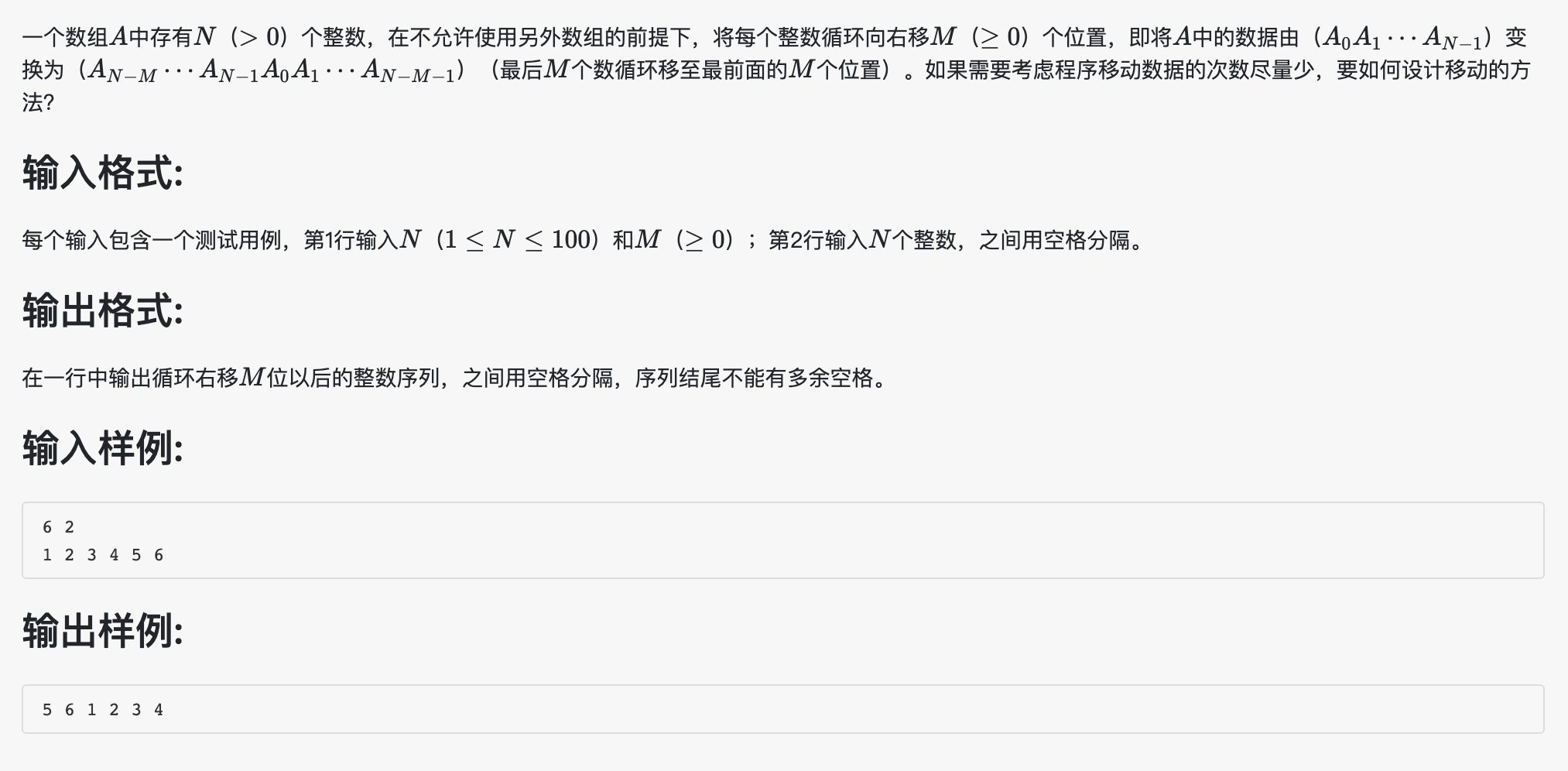#!/usr/bin/env python
# -*-coding:utf-8 -*-
'''
@File    :   1008.py
@Time    :   2020/03/17 18:22:14
@Author  :   Schiller Xu
@Version :   1.0
@Contact :   schillerxu@qq.com
@Desc    :   None
'''

nums=input().split()
num=int(nums)
n=int(nums)
index=num-1

data=input().split()
array=[int(data[i]) for i in range(num)]

while n>0:
tmp=array[index]
i=index
while i>-1:
array[i]=array[i-1]
i=i-1
array=tmp
n=n-1

for j in range(index):
print(array[j],end=' ')
print(array[index])


### 9、说反话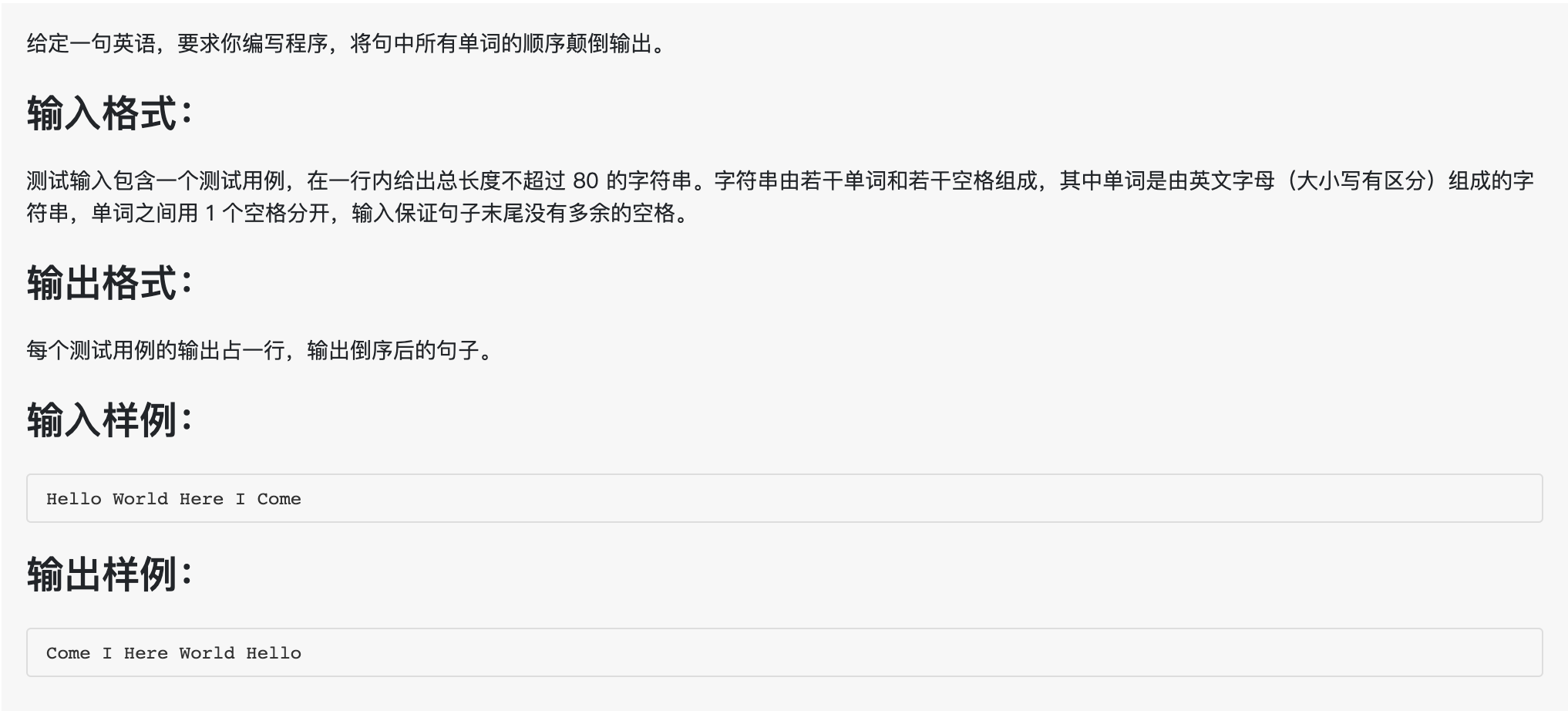#!/usr/bin/env python
# -*-coding:utf-8 -*-
'''
@File    :   1009.py
@Time    :   2020/03/17 19:52:03
@Author  :   Schiller Xu
@Version :   1.0
@Contact :   schillerxu@qq.com
@Desc    :   None
'''

words=input().split()
words.reverse()
index=len(words)-1

for i in range(index):
print(words[i],end=' ')
print(words[index])


### 10、一元多项式求导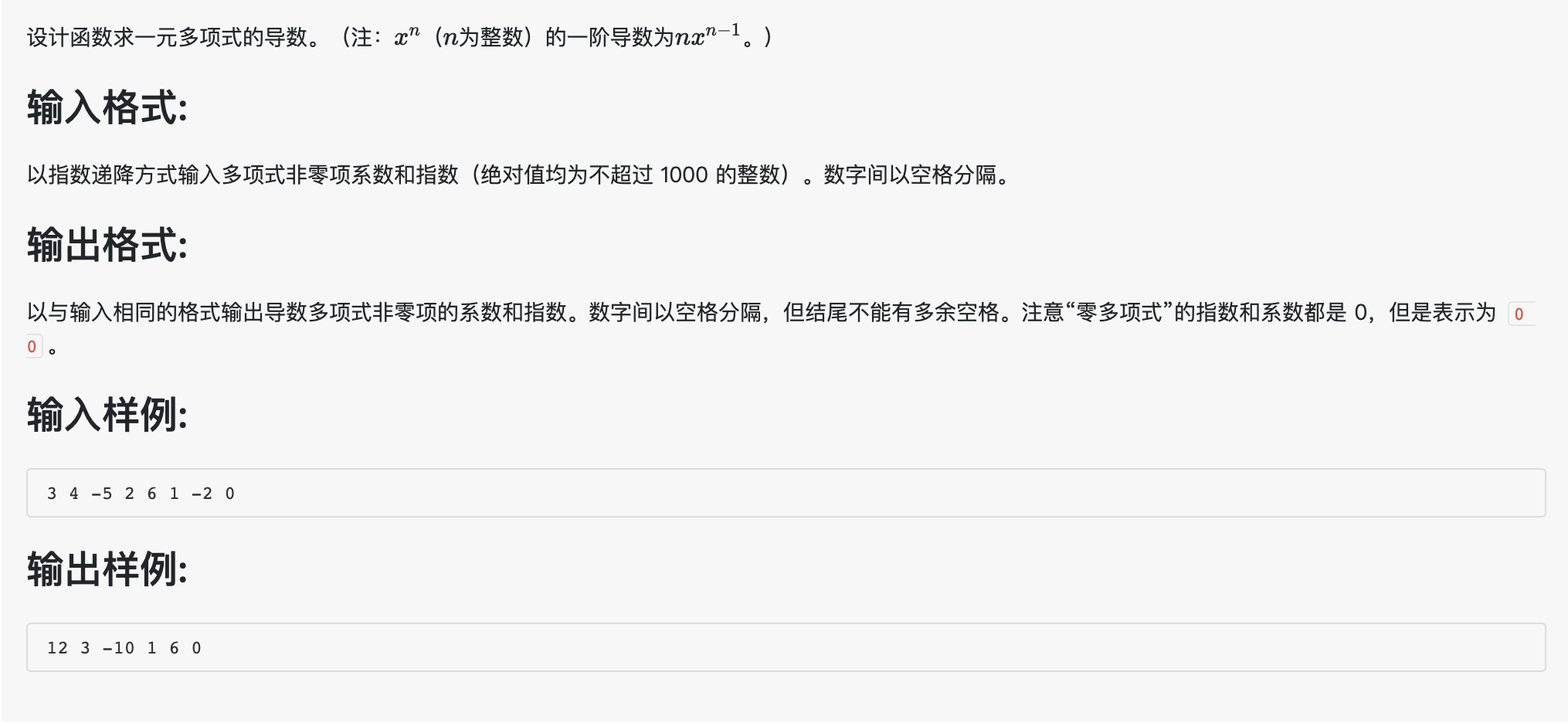#!/usr/bin/env python
# -*-coding:utf-8 -*-
'''
@File    :   1010.py
@Time    :   2020/03/17 20:11:54
@Author  :   Schiller Xu
@Version :   1.0
@Contact :   schillerxu@qq.com
@Desc    :   None
'''

list1 = input().split()
data = [int(i) for i in list1]
index = len(data)-1
result = []

for i in range(0,index,2):
a=data[i]
b=data[i+1]
if b!=0:
result.append(a*b)
result.append(b-1)

lens=len(result)-1
if lens>0:
for j in range(lens):
print(result[j], end=' ')
print(result[lens])
else:
print("0 0")


### 11、A+B 和 C

4
1 2 3
2 3 4
2147483647 0 2147483646
0 -2147483648 -2147483647

Case #1: false
Case #2: true
Case #3: true
Case #4: false

#!/usr/bin/env python
# -*-coding:utf-8 -*-
'''
@File    :   1011.py
@Time    :   2020/03/18 21:22:19
@Author  :   Schiller Xu
@Version :   1.0
@Contact :   schillerxu@qq.com
@Desc    :   None
'''

n=int(input())
data=[input() for i in range(n)]

for i in range(n):
numbers=data[i].split()
f=int(numbers)
s=int(numbers)
t=int(numbers)
print(f"Case #{i+1}:",end=' ')
if f+s>t:
print('true')
else:
print('false')


### 12、数字分类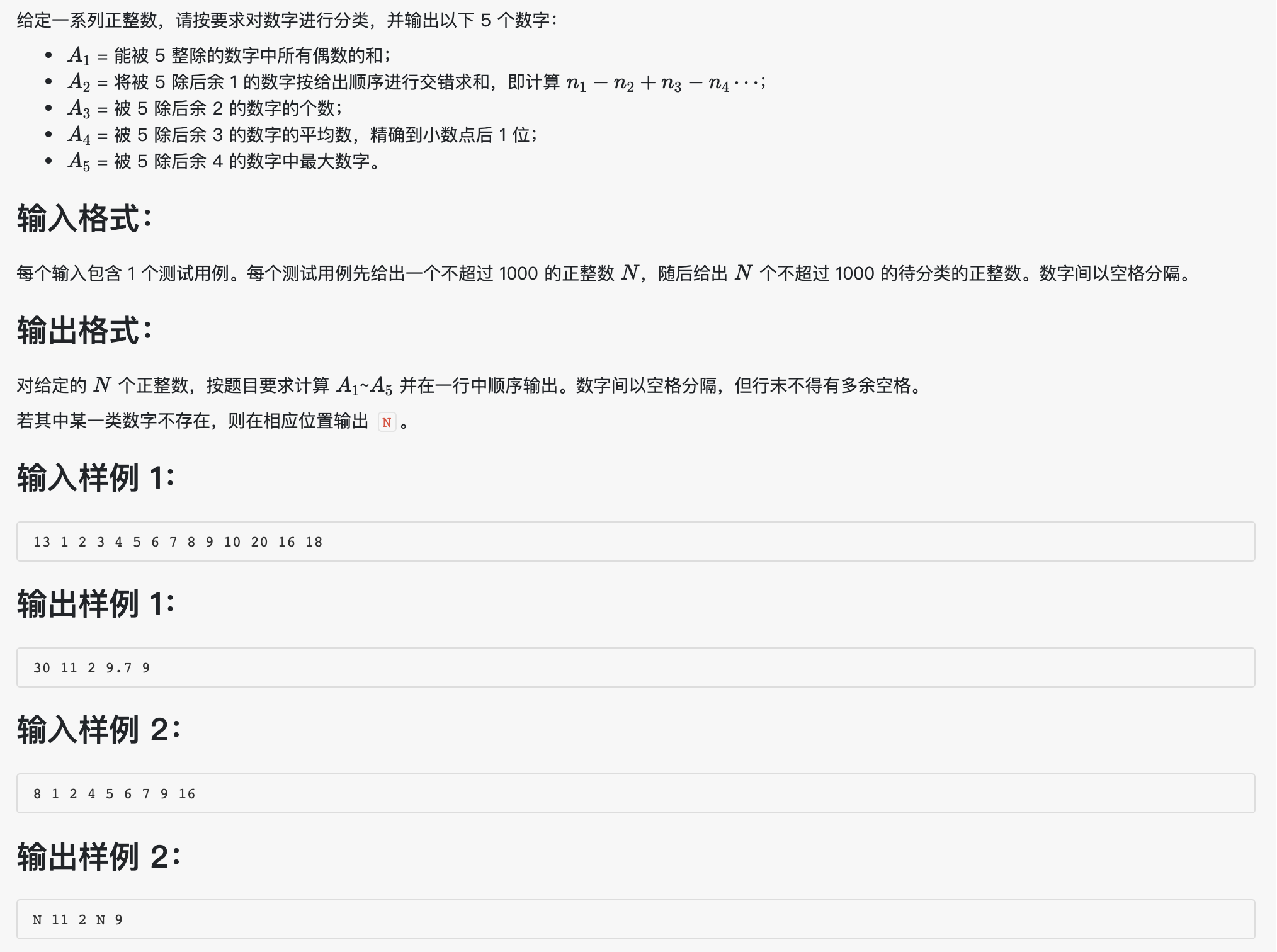#!/usr/bin/env python
# -*-coding:utf-8 -*-
'''
@File    :   1012.py
@Time    :   2020/03/18 21:39:23
@Author  :   Schiller Xu
@Version :   1.0
@Contact :   schillerxu@qq.com
@Desc    :   None
'''

string=input().split()
n=int(string)
numbers=[int(string[i]) for i in range(1,n+1)]

a1=[]
a2=[]
a3=[]
a4=[]
a5=[]

for i in numbers:
if i%10==0:
a1.append(i)
if i%5==1:
a2.append(i)
if i%5==2:
a3.append(i)
if i%5==3:
a4.append(i)
if i%5==4:
a5.append(i)
s=0
if len(a1)==0:
print('N',end=' ')
else:
for i in a1:
s=s+i
print(s,end=' ')

s=0
lens=len(a2)
if lens==0:
print('N',end=' ')
else:
for i in range(lens):
s=s+(-1)**i*a2[i]
print(s,end=' ')

lens=len(a3)
if lens==0:
print('N',end=' ')
else:
print(lens,end=' ')

s=0
lens=len(a4)
if lens==0:
print('N',end=' ')
else:
for i in a4:
s=s+i
m=round(s/lens,1)
print(m,end=' ')

lens=len(a5)
if lens==0:
print('N')
else:
a5.sort(reverse=True)
print(a5)


### 13、数素数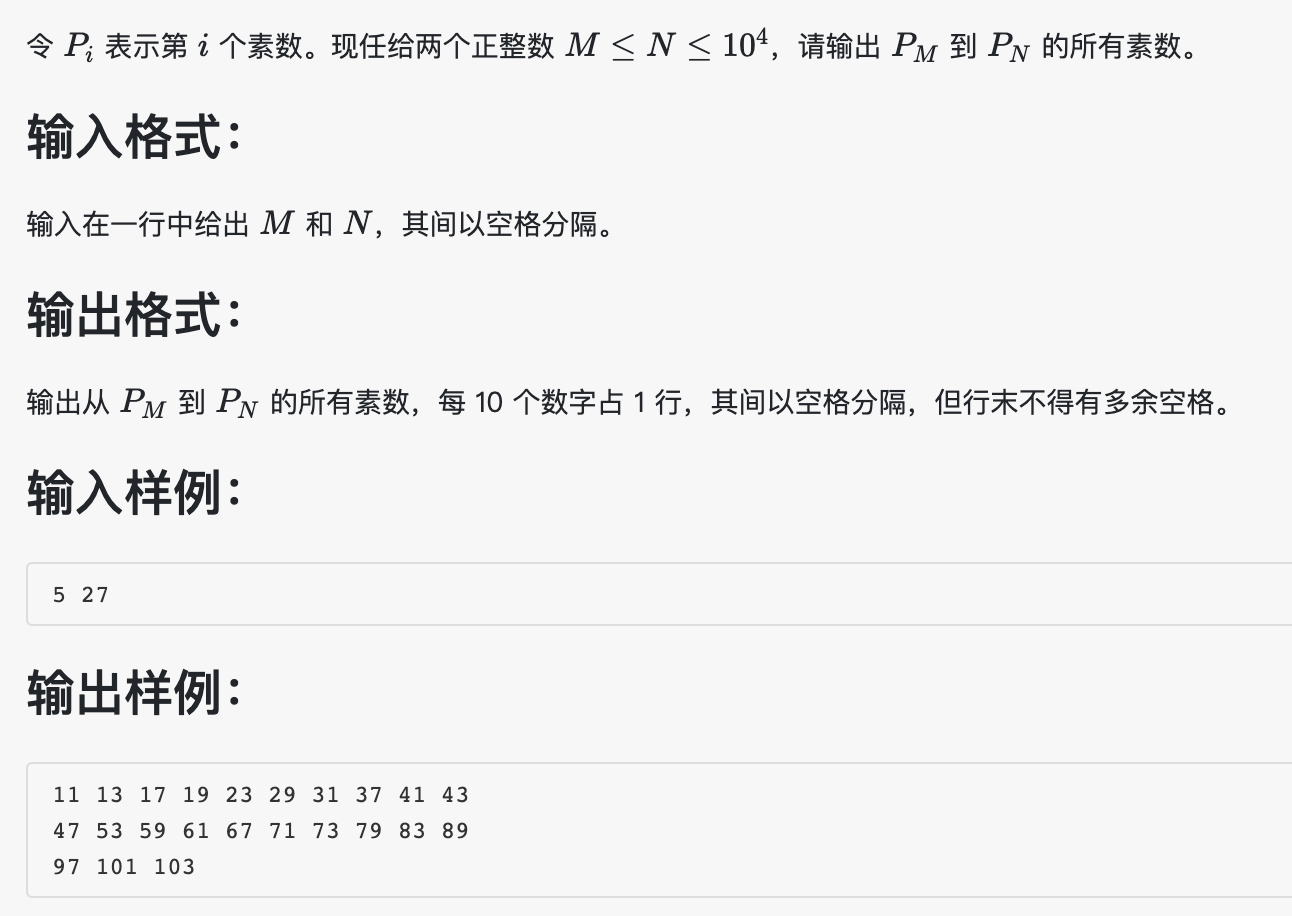#!/usr/bin/env python
# -*-coding:utf-8 -*-
'''
@File    :   1013.py
@Time    :   2020/03/20 22:01:34
@Author  :   Schiller Xu
@Version :   1.0
@Contact :   schillerxu@qq.com
@Desc    :   None
'''

def primes_check(num):
prim = True
sqrt = int(num**0.5)
for i in range(3, sqrt+1):
if num % i == 0:
prim = False
break
return prim

data = input().split()
m = int(data)
n = int(data)
# m=1
# n=10000

if m == 1:
if n==1:
print(2)
else:
print(2, end=' ')
prime = 3
i = 2

while i<m:
prime+=2
while not primes_check(prime):
prime+=2
i+=1

while i < n:
if (i-m) % 10 == 9:
print(prime)
else:
print(prime, end=' ')
prime+=2
while not primes_check(prime):
prime += 2
i += 1
if i==n:
print(prime)


#include<iostream>
#include<math.h>
using namespace std;

bool primes_check(int num){
bool prim=true;
int sq = int(sqrt(num))+1;
for(int i=3;i<sq;i++){
if (num % i == 0){
prim = false;
break;
}
}
return prim;
}

int main()
{
int m,n;
cin>>m>>n;
// m=1;
// n=10000;
if(m==1){
if(n==1){
cout<<"2"<<endl;
}
else{
cout<<"2 ";
}
}
//cout<<"m="<<m<<" ;n="<<n<<endl;
int prime = 3;
int i = 2;

while(i<m){
prime+=2;
while(!primes_check(prime))
prime+=2;
i+=1;
}
//cout<<"i="<<i<<endl;
while(i<n){
if((i-m)%10==9){
cout<<prime<<endl;
}else{
cout<<prime<<" ";
}
//cout<<"prime="<<prime<<"; i="<<i<<endl;
prime+=2;
while(!primes_check(prime)){
prime+=2;
}
i+=1;
}
if(i==n){
cout<<prime<<endl;
}
return 0;
}


### 14、福尔摩斯的约会

3485djDkxh4hhGE
2984akDfkkkkggEdsb
s&hgsfdk
d&Hyscvnm

THU 14:04

#!/usr/bin/env python
# -*-coding:utf-8 -*-
'''
@File    :   1014.py
@Time    :   2020/03/22 13:17:05
@Author  :   Schiller Xu
@Version :   1.0
@Contact :   schillerxu@qq.com
@Desc    :   None
'''

a1=input()
a2=input()
a3=input()
a4=input()
# a1='3485djDkxh4hhGE'
# a2='2984akDfkkkkggEdsb'
# a3='s&hgsfdk'
# a4='d&Hyscvnm'
week=['MON','TUE','WED','THU','FRI','SAT','SUN']
time=[]

lens=min(len(a1),len(a2))

for i in range(lens):
if a1[i]==a2[i] and ('A'<=a1[i]<='G'):
asc=ord(a1[i])-65
time.append(week[asc])
break

for j in range(i+1,lens):
if a1[j]==a2[j] and ('A'<=a1[j]<='N'):
asc=str(ord(a1[j])-55)
time.append(asc)
break
elif a1[j]==a2[j] and ('0'<=a1[j]<='9'):
asc='0'+a1[j]
time.append(asc)
break

for k in range(min(len(a3),len(a4))):
if (('A'<=a3[k]<='Z') or ('a'<=a3[k]<='z')) and a3[k]==a4[k]:
if k<10:
mini='0'+str(k)
else:
mini=str(k)
time.append(mini)
break

print(time+" "+time+":"+time)


### 15、德才论（有超时）

14 60 80
10000001 64 90
10000002 90 60
10000011 85 80
10000003 85 80
10000004 80 85
10000005 82 77
10000006 83 76
10000007 90 78
10000008 75 79
10000009 59 90
10000010 88 45
10000012 80 100
10000013 90 99
10000014 66 60

12
10000013 90 99
10000012 80 100
10000003 85 80
10000011 85 80
10000004 80 85
10000007 90 78
10000006 83 76
10000005 82 77
10000002 90 60
10000014 66 60
10000008 75 79
10000001 64 90

#!/usr/bin/env python
# -*-coding:utf-8 -*-
'''
@File    :   1015.py
@Time    :   2020/03/23 12:50:12
@Author  :   Schiller Xu
@Version :   1.0
@Contact :   schillerxu@qq.com
@Desc    :   None
'''

data = input().split()
n = int(data)
l = int(data)
h = int(data)

grades = [input().split() for i in range(n)]

result1 = []
result2 = []
result3 = []
result4 = []

if d >= l and c >= l:
if d >= h and c >= h:
elif d >= h > c:
elif c <= d < h:
else:

m = len(result1)+len(result2)+len(result3)+len(result4)
print(m)
for i in result1+result2+result3+result4:
print(i+' '+i+' '+i)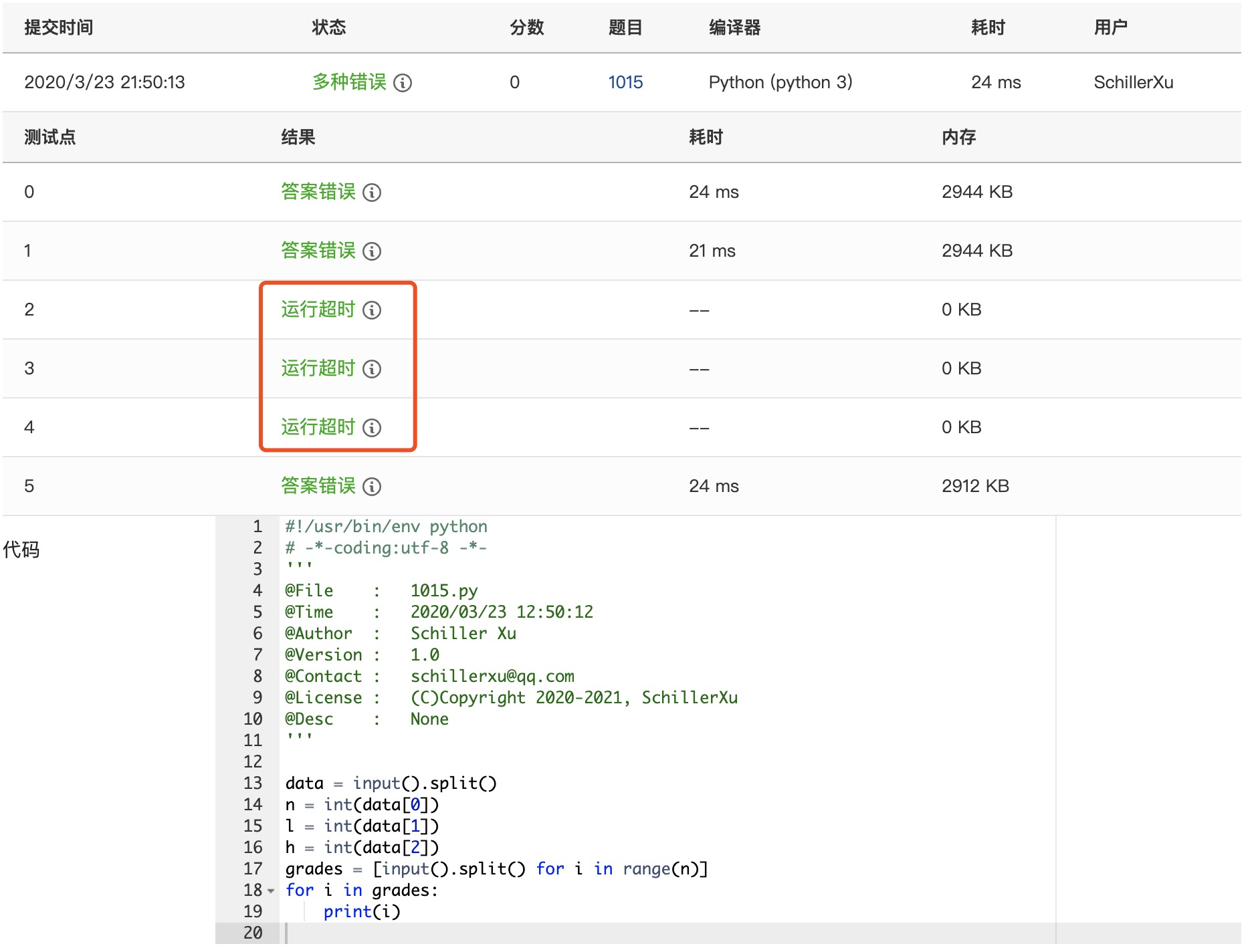python用来刷算法，遇到大点的测试数据确实容易吃亏，后面就不怎么纠结运行超时了。

### 16、部分A+B

3862767 6 13530293 3

399

3862767 1 13530293 8

0

#!/usr/bin/env python
# -*-coding:utf-8 -*-
'''
@File    :   1016.py
@Time    :   2020/03/24 11:35:52
@Author  :   Schiller Xu
@Version :   1.0
@Contact :   schillerxu@qq.com
@Desc    :   None
'''

data=input().split()
a=data
da=data
b=data
db=data

num_a=a.count(da)
num_b=b.count(db)

if num_a==0:
pa=0
else:
pa=int(da*num_a)

if num_b==0:
pb=0
else:
pb=int(db*num_b)

sum=pa+pb
print(sum)


### 17、A除以B

123456789050987654321 7

17636684150141093474 3

#!/usr/bin/env python
# -*-coding:utf-8 -*-
'''
@File    :   1017.py
@Time    :   2020/03/24 12:00:18
@Author  :   Schiller Xu
@Version :   1.0
@Contact :   schillerxu@qq.com
@Desc    :   None
'''

data=input().split()
num=data
d=int(data)

result=str(int(int(num)/d))
re=str(int(num)%d)

for i in range(1,len(num)):
result+=str(int(int(re+num[i])/d))
re=str(int(re+num[i])%d)

if result=='0'and len(result)>1:
result=result[1:]
print(result+' '+re)


### 18、锤子剪刀布（有超时）10
C J
J B
C B
B B
B C
C C
C B
J B
B C
J J

5 3 2
2 3 5
B B

#!/usr/bin/env python
# -*-coding:utf-8 -*-
'''
@File    :   1018.py
@Time    :   2020/03/24 12:51:44
@Author  :   Schiller Xu
@Version :   1.0
@Contact :   schillerxu@qq.com
@Desc    :   None
'''

n = int(input())
data = [input() for i in range(n)]
w = 0
f = 0
# 甲出剪刀石头布赢的次数
w_c = 0
w_j = 0
w_b = 0
# 乙出剪刀石头布赢的次数
f_c = 0
f_j = 0
f_b = 0

for i in data:
if i == 'C':
if i == 'J':
w += 1
w_c += 1
elif i == 'B':
f += 1
f_b += 1
elif i == 'J':
if i == 'B':
w += 1
w_j += 1
elif i == 'C':
f += 1
f_c += 1
else:
if i == 'C':
w += 1
w_b += 1
elif i == 'J':
f += 1
f_j += 1

p = n-w-f
print(f"{w} {p} {f}")
print(f"{f} {p} {w}")

if w_b >= w_c:
if w_b >= w_j:
print('B', end=' ')
else:
print('J', end=' ')
else:
if w_c >= w_j:
print('C', end=' ')
else:
print('J', end=' ')

if f_b >= f_c:
if f_b >= f_j:
print('B')
else:
print('J')
else:
if f_c >= f_j:
print('C')
else:
print('J')


### 19、数字黑洞

7766 - 6677 = 1089
9810 - 0189 = 9621
9621 - 1269 = 8352
8532 - 2358 = 6174
7641 - 1467 = 6174
… …

6767

7766 - 6677 = 1089
9810 - 0189 = 9621
9621 - 1269 = 8352
8532 - 2358 = 6174

2222

2222 - 2222 = 0000

#!/usr/bin/env python
# -*-coding:utf-8 -*-
'''
@File    :   1019.py
@Time    :   2020/03/25 13:49:51
@Author  :   Schiller Xu
@Version :   1.0
@Contact :   schillerxu@qq.com
@Desc    :   None
'''

string=input()
lens=len(string)
if lens!=4:
string='0'*(4-lens)+string
nums=[i for i in string]

if nums==nums==nums==nums:
print(string+' - '+string+' = 0000')
exit(0)

nums.sort()
str_b=nums+nums+nums+nums
str_l=nums+nums+nums+nums
b=int(str_b)
l=int(str_l)
i=b-l
res=str(i)
if len(res)!=4:
res='0'*(4-len(res))+res
print(f'{str_b} - {str_l} = {res}')

while i!=6174:
# s=str(i)
# if len(s)!=4:
#     s='0'*(4-len(s))+s
s=res
nums.clear()
nums=[j for j in s]
nums.sort()
str_b=nums+nums+nums+nums
str_l=nums+nums+nums+nums
b=int(str_b)
l=int(str_l)
i=b-l
res=str(i)
if len(res)!=4:
res='0'*(4-len(res))+res
print(f'{str_b} - {str_l} = {res}')


### 20、月饼

3 20
18 15 10
75 72 45

94.50

#!/usr/bin/env python
# -*-coding:utf-8 -*-
'''
@File    :   1020.py
@Time    :   2020/03/25 18:42:16
@Author  :   Schiller Xu
@Version :   1.0
@Contact :   schillerxu@qq.com
@Desc    :   None
'''

nd=input().split()
n=int(nd)
d=float(nd)

stock=input().split()
prices=input().split()

# data=[[int(i),int(j)] for i,j in zip(stock,prices)]
# data = list(filter(lambda x: x!=0, data))
data=[]
for i in range(n):
x=[]
x.append(float(stock[i]))
x.append(float(prices[i]))
data.append(x)

data.sort(key=lambda x: x/x,reverse=True)

#print(data)

sum=0
for i in data:
if d>i:
d=d-i
sum+=i
else:
r=d/i*i
sum+=r
break

# str_sum=str(sum).split('.')
# if len(str_sum)==1:
#     str_sum.append('00')
# elif len(str_sum)==1:
#     str_sum+='0'

# print(str_sum+'.'+str_sum)
print('{:.2f}'.format(sum))


format用来控制格式比较好用，可参考：
python中的format函数

### 21、个位数统计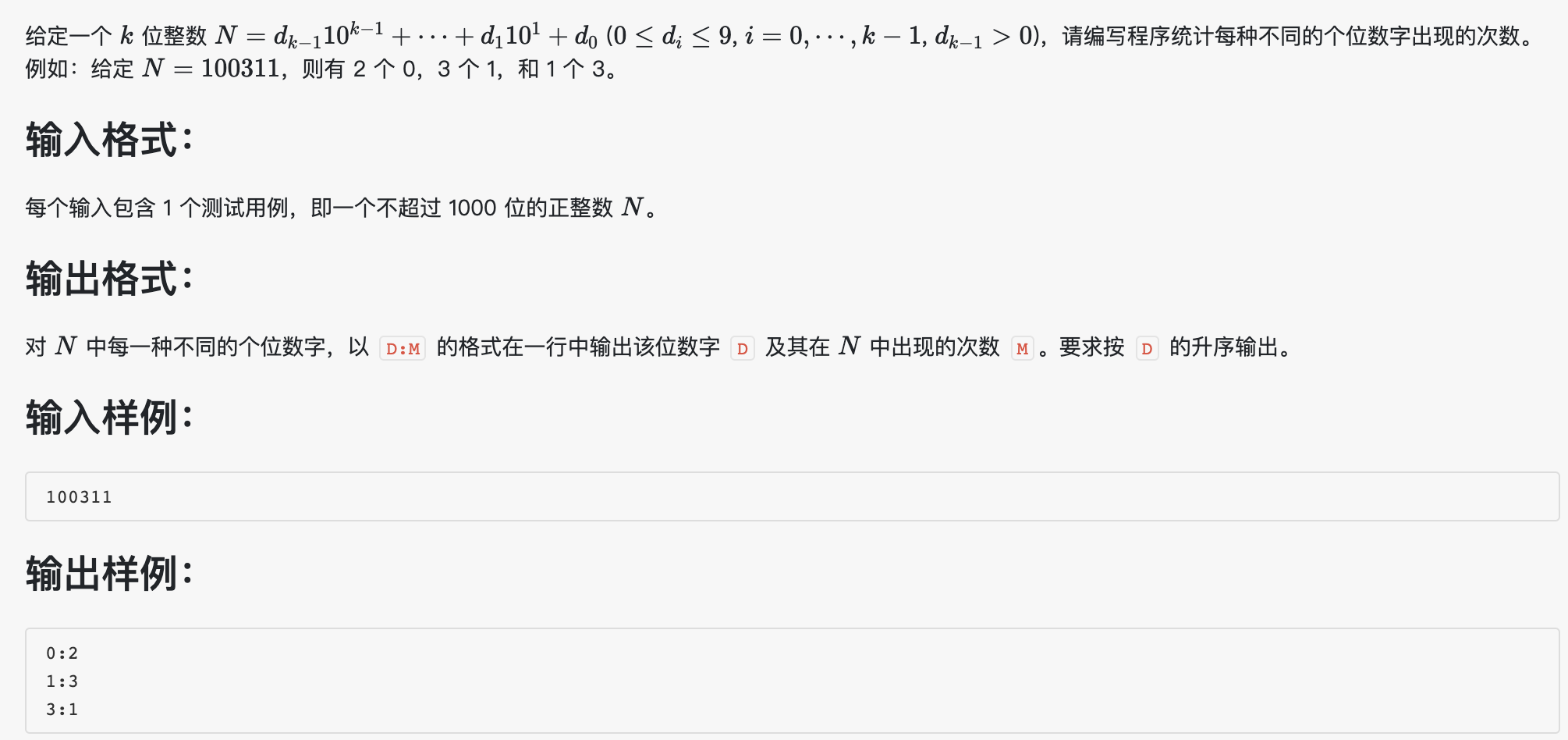#!/usr/bin/env python
# -*-coding:utf-8 -*-
'''
@File    :   1021.py
@Time    :   2020/03/26 13:00:46
@Author  :   Schiller Xu
@Version :   1.0
@Contact :   schillerxu@qq.com
@Desc    :   None
'''

num=input()
result1=[]
result2=[]
for i in num:
if i not in result1:
result1.append(i)
result2.append(1)
else:
n=result1.index(i)
result2[n]+=1

result=[[i,j] for i,j in zip(result1,result2)]
result.sort(key=lambda x: x)

for i in result:
print(i+':'+str(i))


### 22、D进制的A+B

123 456 8

1103

#!/usr/bin/env python
# -*-coding:utf-8 -*-
'''
@File    :   1022.py
@Time    :   2020/03/26 13:23:53
@Author  :   Schiller Xu
@Version :   1.0
@Contact :   schillerxu@qq.com
@Desc    :   None
'''

data=input().split()

a=int(data)
b=int(data)
sum=a+b
c=int(data)

if sum==0:
print('0')
exit()

result=[]
while sum!=0:
result.append(str(sum%c))
sum=int(sum/c)

result.reverse()
for i in result:
print(i,end='')


### 23、组个最小数

2 2 0 0 0 3 0 0 1 0

10015558

#!/usr/bin/env python
# -*-coding:utf-8 -*-
'''
@File    :   1023.py
@Time    :   2020/03/26 13:55:20
@Author  :   Schiller Xu
@Version :   1.0
@Contact :   schillerxu@qq.com
@Desc    :   None
'''

nums=input().split()
num_str=''
for i in range(10):
num_str+=str(i)*int(nums[i])
data=list(num_str)

data.sort()

for i in range(len(data)):
if data[i]!='0':
break

if i!=0:
swap=data[i]
data[i]=data
data=swap

for j in data:
print(j,end='')


### 24、科学计数法

+1.23400E-03

0.00123400

-1.2E+10

-12000000000

#!/usr/bin/env python
# -*-coding:utf-8 -*-
'''
@File    :   test.py
@Time    :   2020/03/27 11:52:27
@Author  :   Schiller Xu
@Version :   1.0
@Contact :   schillerxu@qq.com
@Desc    :   None
'''

data = input().split('E')
sign = data  # 数字部分符号
s = data + data[3:]  # 去掉符号和小数点的数字部分
e = data  # E后的部分

if int(e) < 0:
x = int(e) + 1
s = '0' + '.' + '0' * (-x) + s
else:
x = int(e) + 1
if x >= len(s):
s = s + '0' * (x - len(s))
else:
s = s[0:x] + '.' + s[x:]

if sign == '-':
s = '-' + s
print(s)


\$ python 1024.py
+0.001E+1
00.01


### 25、反转链表（有超时）

00100 6 4
00000 4 99999
00100 1 12309
68237 6 -1
33218 3 00000
99999 5 68237
12309 2 33218

00000 4 33218
33218 3 12309
12309 2 00100
00100 1 99999
99999 5 68237
68237 6 -1

#!/usr/bin/env python
# -*-coding:utf-8 -*-
'''
@File    :   test.py
@Time    :   2020/04/01 10:24:29
@Author  :   Schiller Xu
@Version :   1.0
@Contact :   schillerxu@qq.com
@Desc    :   None
'''

data=input().split()

first=data
n=int(data)
k=int(data)

nodes=[input().split() for i in range(n)]

r=[]
result=[]

while first!='-1':
for node in nodes:
if node==first:
first=node
r.append(node)
break

for i in range(0,n,k):
if len(r[i:i+k])==k:
temp=r[i:i+k]
temp.reverse()
result+=temp
else:
result+=r[i:i+k]
break

#range(n-1)会有一个错误
for i in range(len(result)-1):
result[i]=result[i+1]
result[-1]='-1'

for r in result:
print("{0} {0} {0}".format(r))


1、链表生成后需要调整“Next”字段；
2、调整链表字段时，for循环代码（注释处）改为for i in range(n-1)除超时外还有一个错误，暂时还未解决；如果了解，望留言，感谢！

### 26、程序运行时间

123 4577973

12:42:59

#!/usr/bin/env python
# -*-coding:utf-8 -*-
'''
@File    :   1026.py
@Time    :   2020/04/04 16:23:35
@Author  :   Schiller Xu
@Version :   1.0
@Contact :   schillerxu@qq.com
@Desc    :   None
'''

data=input().split()

c1=int(data)
c2=int(data)
clk=100

second=str((c2-c1)/clk)

seconds=second.split('.')
if len(seconds)>=2 and seconds>='5':
num=int(seconds)+1
else:
num=int(seconds)

s=num%60
num=int(num/60)
m=num%60
h=int(num/60)
print("{0:02}:{1:02}:{2:02}".format(h,m,s))


### 27、打印沙漏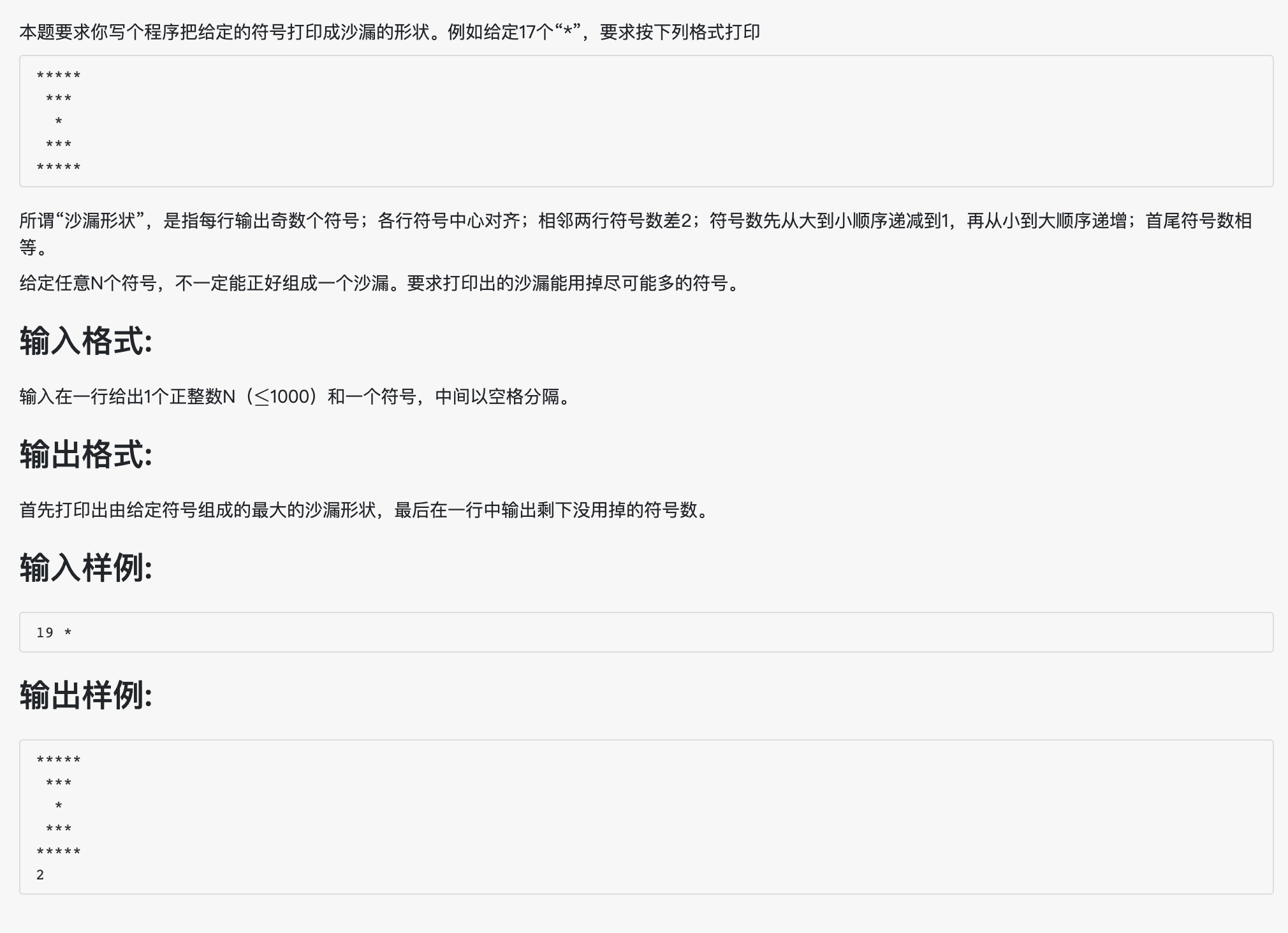#!/usr/bin/env python
# -*-coding:utf-8 -*-
'''
@File    :   1027.py
@Time    :   2020/04/04 17:46:32
@Author  :   Schiller Xu
@Version :   1.0
@Contact :   schillerxu@qq.com
@Desc    :   None
'''

data=input().split()
n=int(data)
s=data

n=n-1
i=1

while n>=2*(i+2):
i=i+2
n=n-i*2

j=i
k=0
while j>0:
line=' '*k+s*j
print(line)
j=j-2
k=k+1

for j in range(3,i+1,2):
k=int((i-j)/2)
line=' '*k+s*j
print(line)
print(n)


### 28、人口普查（有超时）

5
John 2001/05/12
Tom 1814/09/06
Ann 2121/01/30
James 1814/09/05
Steve 1967/11/20

3 Tom John

#!/usr/bin/env python
# -*-coding:utf-8 -*-
'''
@File    :   1028.py
@Time    :   2020/04/04 19:40:11
@Author  :   Schiller Xu
@Version :   1.0
@Contact :   schillerxu@qq.com
@Desc    :   None
'''

n=int(input())

data=[input().split() for i in range(n)]

# for info in data[:]:
#     t=info.split('/')
#     s=int(t+t+t)
#     if s<18140906 or s>20140906:
#         data.remove(info)
#     else:
#         info.append(s)

for i in range(len(data)-1,-1,-1):
t=data[i].split('/')
s=int(t+t+t)
if s<18140906 or s>20140906:
data.pop(i)
else:
data[i].append(s)

data.sort(key=lambda x: x[-1])
lens=len(data)
if lens==0:
print(0)
else:
print("{} {} {}".format(lens,data,data[-1]))


### 29、旧键盘

7_This_is_a_test
_hs_s_a_es

7TI

#!/usr/bin/env python
# -*-coding:utf-8 -*-
'''
@File    :   1029.py
@Time    :   2020/04/05 20:54:17
@Author  :   Schiller Xu
@Version :   1.0
@Contact :   schillerxu@qq.com
@Desc    :   None
'''

a=list(input())
b=input()

for char in b:
for i in range(len(a)-1,-1,-1):
if a[i]==char:
a.pop(i)

for i in range(len(a)):
if 'a'<=a[i]<='z':
a[i]=chr(ord(a[i])-32)

for i in range(len(a)-1):
for j in range(len(a)-1,i,-1):
if a[i]==a[j]:
a.pop(j)

print("".join(a))


### 30、完美数列

10 8
2 3 20 4 5 1 6 7 8 9

8

#!/usr/bin/env python
# -*-coding:utf-8 -*-
'''
@File    :   1030.py
@Time    :   2020/04/06 11:43:20
@Author  :   Schiller Xu
@Version :   1.0
@Contact :   schillerxu@qq.com
@Desc    :   None
'''

data=input().split()
n=int(data)
p=int(data)

nums= sorted(map(int, input().split()))
m=1

# 以下面的测试数据说明算法：
# 输入：
# 8 2
# 1 2 5 6 13 14 30 40
# m始终记录的是完美数列最大的数字个数，以1作为最小数组成的数列数字个数是2(m)，那以2作为最小数组成的数列数字个数要想大于2，只需要比较2*2(nums[i]*p)和6(nums[i+m])即可。如果nums[i+m]不满足完美数列的条件，就说明以2组成的数列数字个数<=m，m不操作；如果nums[i+m]满足完美数列的条件，m加1。

for i in range(n):
for j in range(i+m,n):
if nums[i]*p>=nums[j]:
m+=1
else:
break

print(m)


### 31、查验身份证

Z：0 1 2 3 4 5 6 7 8 9 10
M：1 0 X 9 8 7 6 5 4 3 2

4
320124198808240056
12010X198901011234
110108196711301866
37070419881216001X

12010X198901011234
110108196711301866
37070419881216001X

2
320124198808240056
110108196711301862

All passed

#!/usr/bin/env python
# -*-coding:utf-8 -*-
'''
@File    :   1031.py
@Time    :   2020/04/08 16:29:48
@Author  :   Schiller Xu
@Version :   1.0
@Contact :   schillerxu@qq.com
@Desc    :   None
'''

n=int(input())

data = [input() for i in range(n)]
w=[7,9,10,5,8,4,2,1,6,3,7,9,10,5,8,4,2]
check=['1', '0', 'X', '9', '8', '7', '6', '5', '4', '3', '2']

i=0

for id in data:
sum=0
c=True
for j in range(17):
if '0'<=id[j]<='9':
sum+=w[j]*int(id[j])
else:
c=False
break
sum=sum%11
if id[-1]!=check[sum] or c==False:
i+=1
print(id)

if i==0:
print('All passed')


### 32、挖掘机技术哪家强（有超时）

6
3 65
2 80
1 100
2 70
3 40
3 0

2 150

#!/usr/bin/env python
# -*-coding:utf-8 -*-
'''
@File    :   1032.py
@Time    :   2020/04/08 17:02:03
@Author  :   Schiller Xu
@Version :   1.0
@Contact :   schillerxu@qq.com
@Desc    :   None
'''

n=int(input())
school=[]
result=[]

for i in range(n):
data=input().split()
if data in school:
p=school.index(data)
else:
school.append(data)

index=0
index=i

print(school[index],end=' ')
print(m)


### 33、旧键盘打字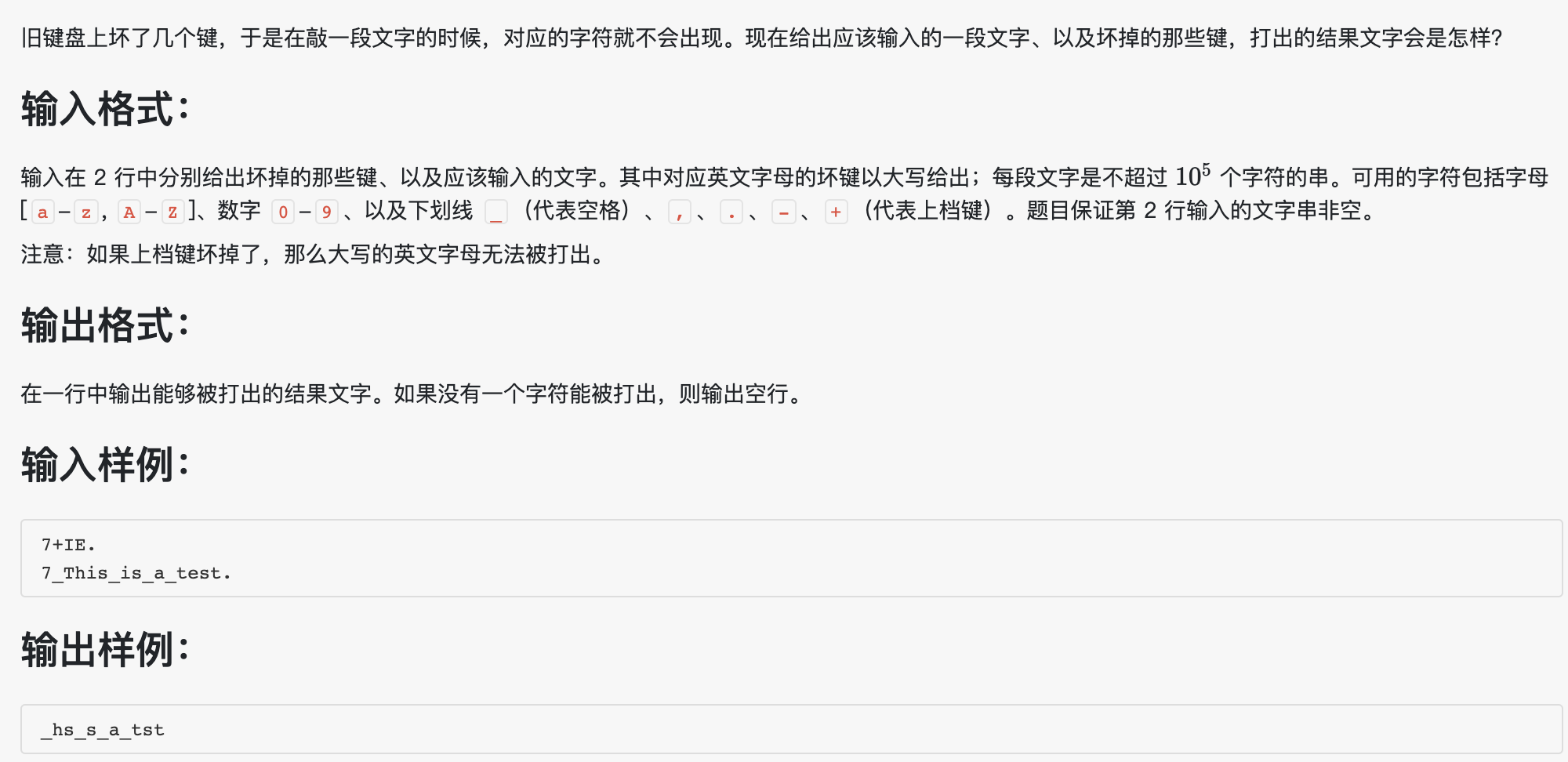#!/usr/bin/env python
# -*-coding:utf-8 -*-
'''
@File    :   1033.py
@Time    :   2020/04/10 13:20:06
@Author  :   Schiller Xu
@Version :   1.0
@Contact :   schillerxu@qq.com
@Desc    :   None
'''

#a=list(input())
a=input()
b=input()
sign=0

if '+' in a:
sign=1

len_a=len(a)
for i in a:
if 'A'<=i<='Z':
a=a+i.lower()

for i in b:
if i  not in a and not (sign==1 and 'A'<=i<='Z'):
print(i,end='')

#print(''.join(b))


### 34、有理数四则运算

2/3 -4/2

2/3 + (-2) = (-1 1/3)
2/3 - (-2) = 2 2/3
2/3 * (-2) = (-1 1/3)
2/3 / (-2) = (-1/3)

5/3 0/6

1 2/3 + 0 = 1 2/3
1 2/3 - 0 = 1 2/3
1 2/3 * 0 = 0
1 2/3 / 0 = Inf

#!/usr/bin/env python
# -*-coding:utf-8 -*-
'''
@File    :   1034.py
@Time    :   2020/04/13 09:06:20
@Author  :   Schiller Xu
@Version :   1.0
@Contact :   schillerxu@qq.com
@Desc    :   None
'''

from fractions import Fraction

def print_num(number):
a=int(number)
#b=Fraction(number-a).limit_denominator()
b=number-a
if a==0:
if b!=0:
result=f'{b}'
else:
result='0'
else:
if b==0:
result=f'{a}'
else:
b=abs(b)
result=f'{a} {b}'
if number<0:
result=f'({result})'

return result

data=input().split()
#nums=data.split('/')+data.split('/')
nums=[int(i) for i in data.split('/')+data.split('/')]

# num1=int(nums)/int(nums)
# num2=int(nums)/int(nums)
num1=Fraction(nums,nums)
num2=Fraction(nums,nums)
p_num1=print_num(num1)
p_num2=print_num(num2)

sum=num1+num2
print(p_num1+' + '+p_num2+' = '+print_num(sum))

de=num1-num2
print(p_num1+' - '+p_num2+' = '+print_num(de))

mul=num1*num2
print(p_num1+' * '+p_num2+' = '+print_num(mul))

if num2==0:
print(p_num1+' / '+p_num2+' = Inf')
else:
di=num1/num2
print(p_num1+' / '+p_num2+' = '+print_num(di))


### 35、插入与归并

10
3 1 2 8 7 5 9 4 6 0
1 2 3 7 8 5 9 4 6 0

Insertion Sort
1 2 3 5 7 8 9 4 6 0

10
3 1 2 8 7 5 9 4 0 6
1 3 2 8 5 7 4 9 0 6

Merge Sort
1 2 3 8 4 5 7 9 0 6

#!/usr/bin/env python
# -*-coding:utf-8 -*-
'''
@File    :   1035.py
@Time    :   2020/04/19 20:47:01
@Author  :   Schiller Xu
@Version :   1.0
@Desc    :   None
'''

n=int(input())
data=[int(i) for i in input().split()]
num=[int(i) for i in input().split()]

p=2
while p<n:
c=[]
for i in range(0,n,p):
c=c+sorted(data[i:i+p])
if c==num:
break
#print(c)
p=p*2

if p<n:
print("Merge Sort")
p=p*2
c=[]
for i in range(0,n,p):
c=c+sorted(data[i:i+p])
for j in range(n-1):
print(c[j],end=' ')
print(c[-1])
else:
print("Insertion Sort")
for i in range(n):
if num[i]>num[i+1]:
break
i=i+2
c=[]
c=sorted(num[0:i])+num[i:]
for j in range(n-1):
print(c[j],end=' ')
print(c[-1])


### 36、跟奥巴马一起编程

10 a

aaaaaaaaaa
a        a
a        a
a        a
aaaaaaaaaa

#!/usr/bin/env python
# -*-coding:utf-8 -*-
'''
@File    :   1036.py
@Time    :   2020/04/20 15:13:26
@Author  :   Schiller Xu
@Version :   1.0
@Desc    :   None
'''

data=input().split()
n=int(data)
sign=data

#cow=round(n/2)
if n%2==0:
cow=n//2
else:
cow=n//2+1
print(sign*n)
for i in range(cow-2):
print(sign+' '*(n-2)+sign)
print(sign*n)


### 37、在霍格沃茨找零钱

10.16.27 14.1.28

3.2.1

14.1.28 10.16.27

-3.2.1

#!/usr/bin/env python
# -*-coding:utf-8 -*-
'''
@File    :   1037.py
@Time    :   2020/04/20 15:30:02
@Author  :   Schiller Xu
@Version :   1.0
@Desc    :   None
'''

data=input().split()
p_list=[int(i) for i in data.split('.')]
a_list=[int(i) for i in data.split('.')]

p=p_list*17*29+p_list*29+p_list
a=a_list*17*29+a_list*29+a_list
result=a-p

sign=''
if result<0:
sign='-'
result=-result
k=result%29
result=result//29
s=result%17
g=result//17

print(f'{sign}{g}.{s}.{k}')


### 38、统计同成绩学生（有超时）

10
60 75 90 55 75 99 82 90 75 50
3 75 90 88

3 2 0

#!/usr/bin/env python
# -*-coding:utf-8 -*-
'''
@File    :   1038.py
@Time    :   2020/04/20 15:50:24
@Author  :   Schiller Xu
@Version :   1.0
@Desc    :   None
'''

n=int(input())
points=input().split()

for i in range(1,len(points)-1):
print(a,end=' ')



### 39、到底买不买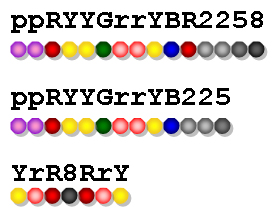ppRYYGrrYBR2258
YrR8RrY

Yes 8

ppRYYGrrYB225
YrR8RrY

No 2

#!/usr/bin/env python
# -*-coding:utf-8 -*-
'''
@File    :   1039.py
@Time    :   2020/04/20 16:09:34
@Author  :   Schiller Xu
@Version :   1.0
@Desc    :   None
'''

a=list(input())
b=input()
num=len(a)-len(b)

i=0

for char in b:
if char in a:
a.remove(char)
else:
i+=1

if i==0:
print("Yes "+str(num))
else:
print("No "+str(i))


### 40、有几个PAT（有超时）

APPAPT

2

#!/usr/bin/env python
# -*-coding:utf-8 -*-
'''
@File    :   1040.py
@Time    :   2020/04/21 09:40:16
@Author  :   Schiller Xu
@Version :   1.0
@Desc    :   None
'''

s=input()
n=0

for i in range(len(s)-1):
if s[i]=='A':
n=n+s[0:i].count('P')*s[i+1:].count('T')

n=n%1000000007

print(n)


### 41、考试座位号

4
3310120150912233 2 4
3310120150912119 4 1
3310120150912126 1 3
3310120150912002 3 2
2
3 4

3310120150912002 2
3310120150912119 1

#!/usr/bin/env python
# -*-coding:utf-8 -*-
'''
@File    :   1041.py
@Time    :   2020/04/21 13:10:43
@Author  :   Schiller Xu
@Version :   1.0
@Desc    :   None
'''

n=int(input())
data=[input().split() for i in range(n)]
m=int(input())
id=input().split()

for i in range(m):
for j in range(n):
if id[i]==data[j]:
print(data[j]+' '+data[j])
break


### 42、字符统计

This is a simple TEST. There ARE numbers and other symbols 1&2&3…

e 7

#!/usr/bin/env python
# -*-coding:utf-8 -*-
'''
@File    :   1042.py
@Time    :   2020/04/21 13:47:24
@Author  :   Schiller Xu
@Version :   1.0
@Desc    :   None
'''

s=input()
result=[]
chars=[]

for c in s:
if 'a'<=c<='z' or 'A'<=c<='Z':
if c.isupper:
c=c.lower()
else:
continue
if c not in chars:
chars.append(c)
result.append([c,1])
else:
for i in range(len(result)):
if result[i]==c:
result[i]+=1

result.sort(key=lambda x: x)
result.sort(key=lambda x: x,reverse=True)
print(result+' '+str(result))


### 43、输出PATest

redlesPayBestPATTopTeePHPereatitAPPT

PATestPATestPTetPTePePee

#!/usr/bin/env python
# -*-coding:utf-8 -*-
'''
@File    :   1043.py
@Time    :   2020/04/21 14:20:30
@Author  :   Schiller Xu
@Version :   1.0
@Desc    :   None
'''

s=input()
chars=[c for c in 'PATest']
nums_chars=[0 for i in range(len(chars))]
n=0

for c in s:
if c in chars:
i=chars.index(c)
nums_chars[i]+=1
n=n+1

while n>0:
for i in range(len(chars)):
if nums_chars[i]>0:
print(chars[i],end='')
n=n-1
nums_chars[i]=nums_chars[i]-1
print()


### 44、火星数字

• 地球人的 0 被火星人称为 tret。
• 地球人数字 1 到 12 的火星文分别为：jan, feb, mar, apr, may, jun, jly, aug, sep, oct, nov, dec。
• 火星人将进位以后的 12 个高位数字分别称为：tam, hel, maa, huh, tou, kes, hei, elo, syy, lok, mer, jou。

4
29
5
elo nov
tam

hel mar
may
115
13

#!/usr/bin/env python
# -*-coding:utf-8 -*-
'''
@File    :   1044.py
@Time    :   2020/04/21 14:59:34
@Author  :   Schiller Xu
@Version :   1.0
@Desc    :   None
'''

n = int(input())
data = [input() for i in range(n)]

mars1 = ['tret', 'jan', 'feb', 'mar', 'apr', 'may',
'jun', 'jly', 'aug', 'sep', 'oct', 'nov', 'dec']
mars2 = ['tam', 'hel', 'maa', 'huh', 'tou', 'kes',
'hei', 'elo', 'syy', 'lok', 'mer', 'jou']

for i in data:
if i.isnumeric():
l=int(i)
if l//13==0:
print(mars1[l%13])
elif l%13==0:
print(mars2[l//13-1])
else:
print(mars2[l//13-1]+' '+mars1[l%13])
else:
words=i.split()
lens=len(words)
if lens==1:
if i in mars1:
l=mars1.index(words)
else:
l=(mars2.index(words)+1)*13
print(l)
else:
l=(mars2.index(words)+1)*13+mars1.index(words)
print(l)


### 45、快速排序

1 的左边没有元素，右边的元素都比它大，所以它可能是主元；

5
1 3 2 4 5

3
1 4 5

#!/usr/bin/env python
# -*-coding:utf-8 -*-
'''
@File    :   1045.py
@Time    :   2020/04/21 20:32:59
@Author  :   Schiller Xu
@Version :   1.0
@Desc    :   None
'''
# 用正确排序和原始序列对比之后，并不能确定最终结果，
#还需要进一步判定，比如原始序列‘3 2 1’，排序之后‘1 2 3’，
#2在两个序列的位置相同，但2并不是主元。

n = int(input())
data = [int(i) for i in input().split()]
max = 0
result = []

for i in data:
if i > max:
max = i
result.append(i)
else:
for j in range(len(result)-1, -1, -1):
if result[j] > i:
result.pop(j)
else:
break

lens = len(result)
print(lens)
result.sort()
if lens >= 1:
for i in range(lens-1):
print(result[i], end=' ')
print(result[-1])
else:
print()


### 46、划拳

5
8 10 9 12
5 10 5 10
3 8 5 12
12 18 1 13
4 16 12 15

1 2

#!/usr/bin/env python
# -*-coding:utf-8 -*-
'''
@File    :   1046.py
@Time    :   2020/04/22 14:23:26
@Author  :   Schiller Xu
@Version :   1.0
@Desc    :   None
'''

n=int(input())
data=[[int(i) for i in input().split()] for i in range(n)]
j=0
y=0

for i in data:
a=i+i
if a==i and a!=i:
y+=1
elif a==i and a!=i:
j+=1

print(f'{j} {y}')


### 47、编程团体赛

6
3-10 99
11-5 87
102-1 0
102-3 100
11-9 89
3-2 61

11 176

#!/usr/bin/env python
# -*-coding:utf-8 -*-
'''
@File    :   1047.py
@Time    :   2020/04/22 14:34:11
@Author  :   Schiller Xu
@Version :   1.0
@Desc    :   None
'''

n=int(input())
data=[]
for i in range(n):
a=[]
for j in input().split():
if '-' in j:
a=j.split('-')
else:
a.append(int(j))
data.append(a)

name=[]
result=[]
for i in data:
if i not in name:
name.append(i)
result.append([i,i])
else:
j=name.index(i)
result[j]+=i

result.sort(key=lambda x: x)
print(result[-1],result[-1])


### 48、数字加密

1234567 368782971

3695Q8118

#!/usr/bin/env python
# -*-coding:utf-8 -*-
'''
@File    :   1048.py
@Time    :   2020/04/22 15:47:24
@Author  :   Schiller Xu
@Version :   1.0
@Desc    :   None
'''

#B是要加密的数，A是加密要用的数，
#A的位数不变，B的位数如果不够需要补‘0’

data=input().split()
a=data
b=data
#对13取余，结果是0-12
num=['0','1', '2', '3', '4', '5', '6', '7', '8', '9', 'J', 'Q', 'K']

result=''
str2=''

lens_a=len(a)
lens_b=len(b)

if lens_a>=lens_b:
b='0'*(lens_a-lens_b)+b
else:
str2=b[0:lens_b-lens_a]
b=b[lens_b-lens_a:]

a=a[::-1]
b=b[::-1]

for i in range(len(a)):
if i%2==0:
c=(int(a[i])+int(b[i]))%13
result=num[c]+result
else:
d=int(b[i])-int(a[i])
if d<0:
d=d+10
result=str(d)+result

result=str2+result
print(result)


### 49、数列的片段和

​5
​​ 的正整数 N，表示数列中数的个数，第二行给出 N 个不超过 1.0 的正数，是数列中的数，其间以空格分隔。

4
0.1 0.2 0.3 0.4

5.00

#!/usr/bin/env python
# -*-coding:utf-8 -*-
'''
@File    :   1049.py
@Time    :   2020/04/23 14:27:31
@Author  :   Schiller Xu
@Version :   1.0
@Desc    :   None
'''

n=int(input())
data=[float(i) for i in input().split()]
sum=0.0
result=[0 for i in range(n)]
for i in range(n):
result[i]=(n-i)*(1+i)

for i in range(n):
sum+=data[i]*result[i]

print("{:.2f}".format(sum))


### 50、螺旋矩阵

12
37 76 20 98 76 42 53 95 60 81 58 93

98 95 93
42 37 81
53 20 76
58 60 76

#!/usr/bin/env python
# -*-coding:utf-8 -*-
'''
@File    :   1050.py
@Time    :   2020/04/23 14:28:27
@Author  :   Schiller Xu
@Version :   1.0
@Desc    :   None
'''

num=int(input())
data=[int(i) for i in input().split()]
data.sort(reverse=True)
d=num-1
m=num
n=1
for i in range(2,int(num**0.5)+1):
if num%i==0 and (num/i-i)<d:
m=int(num/i)
n=i
d=m-n

result=[[0 for i in range(n)] for i in range(m)]

#k控制data下标，q控制逆向旋转的次数
k=0
q=0
lens=len(data)
while k<lens:
for i in range(q,n-q):
result[q][i]=data[k]
k+=1
if k<lens:
for j in range(1+q,m-q):
result[j][i]=data[k]
k+=1
if k<lens:
for l in range(n-2-q,-1+q,-1):
result[j][l]=data[k]
k+=1
if k<lens:
for p in range(m-2-q,q,-1):
result[p][l]=data[k]
k+=1
q+=1

for s in result:
for i in range(n-1):
print(s[i],end=' ')
print(s[-1])


### 51、复数乘法

2.3 3.5 5.2 0.4

-8.68-8.23i

#!/usr/bin/env python
# -*-coding:utf-8 -*-
'''
@File    :   1051.py
@Time    :   2020/04/26 14:22:55
@Author  :   Schiller Xu
@Version :   1.0
@Desc    :   None
'''

import math

data=input().split()
r1=float(data)
p1=float(data)
r2=float(data)
p2=float(data)

a1=r1*math.cos(p1)
b1=r1*math.sin(p1)
a2=r2*math.cos(p2)
b2=r2*math.sin(p2)

a="{:.2f}".format(a1*a2-b1*b2)
b="{:+.2f}".format(a1*b2+b1*a2)

if float(a)==0:
a='0.00'

if float(b)==0:
b='0.00'

print(f'{a}{b}i')


### 52、卖个萌（非零返回）

[╮][╭][o][][/] [<][>]
[╯][╰][^][-][=][>][<][@][⊙]
[Д][▽][_][ε][^] …
4
1 1 2 2 2
6 8 1 5 5
3 3 4 3 3
2 10 3 9 3

╮(╯▽╰)╭
<(@Д=)/~
o(ε)o
Are you kidding me? @/@

#!/usr/bin/env python
# -*-coding:utf-8 -*-
'''
@File    :   1052.py
@Time    :   2020/04/29 14:23:04
@Author  :   Schiller Xu
@Version :   1.0
@Desc    :   None
'''

#import sys
l=[]
s=''
for i in range(3):
data=input()
d=[]
for j in range(len(data)):
if data[j]=='[' :
s=''
continue

if data[j]==']':
d.append(s)
s+=data[j]
l.append(d)

# n=int(n)
n=int(input())

for i in range(n):
try:
# num=[int(i)-1 for i in s.split()]
num=[int(i)-1 for i in input().split()]
result='{0}({1}{2}{3}){4}'.format(l[num],l[num],l[num],l[num],l[num])
print(result)
except:
print(r'Are you kidding me? @\/@')
#参考：https://blog.csdn.net/qq_42800234/article/details/82756290


### 53、住房空置率

• 在观察期内，若存在超过一半的日子用电量低于某给定的阈值 e，则该住房为“可能空置”；
• 若观察期超过某给定阈值 D 天，且满足上一个条件，则该住房为“空置”。

K E 1 E 2 E_{1}E_{2} E k E_{k}

5 0.5 10
6 0.3 0.4 0.5 0.2 0.8 0.6
10 0.0 0.1 0.2 0.3 0.0 0.8 0.6 0.7 0.0 0.5
5 0.4 0.3 0.5 0.1 0.7
11 0.1 0.1 0.1 0.1 0.1 0.1 0.1 0.1 0.1 0.1 0.1
11 2 2 2 1 1 0.1 1 0.1 0.1 0.1 0.1

40.0% 20.0%
（样例解释：第2、3户为“可能空置”，第4户为“空置”，其他户不是空置。）

#!/usr/bin/env python
# -*-coding:utf-8 -*-
'''
@File    :   1053.py
@Time    :   2020/04/30 10:34:07
@Author  :   Schiller Xu
@Version :   1.0
@Desc    :   None
'''

data=input().split()
n=int(data)
e=float(data)
d=int(data)

may_no=0
no=0

for i in range(n):
data=[float(i) for i in input().split()]
days=0
for i in range(1,len(data)):
if data[i]<e:
days+=1
if d<int(data)<2*days:
no+=1
elif int(data)<=d and int(data)<2*days:
may_no+=1

result='{0:.1%} {1:.1%}'.format(may_no/n,no/n)
print(result)


### 54、求平均值

7
5 -3.2 aaa 9999 2.3.4 7.123 2.35

ERROR: aaa is not a legal number
ERROR: 9999 is not a legal number
ERROR: 2.3.4 is not a legal number
ERROR: 7.123 is not a legal number
The average of 3 numbers is 1.38

2
aaa -9999

ERROR: aaa is not a legal number
ERROR: -9999 is not a legal number
The average of 0 numbers is Undefined

#!/usr/bin/env python
# -*-coding:utf-8 -*-
'''
@File    :   1054.py
@Time    :   2020/04/30 12:29:46
@Author  :   Schiller Xu
@Version :   1.0
@Desc    :   None
'''

n=int(input())
data=input().split()
s=0
l=0

for i in range(len(data)):
try:
a=float(data[i])
if '.' in data[i]:
t=data[i].split('.')
if len(t[-1])>2:
raise Exception
if -1000<=a<=1000:
s+=a
l+=1
else:
raise Exception
except:
print(f'ERROR: {data[i]} is not a legal number')
if l==0:
print('The average of 0 numbers is Undefined')
elif l==1:
print('The average of 1 number is {:.2f}'.format(s))
else:
print('The average of {0} numbers is {1:.2f}'.format(l,s/l))


### 55、集体照

• 每排人数为 N/K（向下取整），多出来的人全部站在最后一排；
• 后排所有人的个子都不比前排任何人矮；
• 每排中最高者站中间（中间位置为 m/2+1，其中 m 为该排人数，除法向下取整）；
• 每排其他人以中间人为轴，按身高非增序，先右后左交替入队站在中间人的两侧（例如5人身高为190、188、186、175、170，则队形为175、188、190、186、170。这里假设你面对拍照者，所以你的左边是中间人的右边）；
• 若多人身高相同，则按名字的字典序升序排列。这里保证无重名。

10 3
Tom 188
Mike 170
Eva 168
Tim 160
Joe 190
Ann 168
Bob 175
Nick 186
Amy 160
John 159

Bob Tom Joe Nick
Ann Mike Eva
Tim Amy John

#!/usr/bin/env python
# -*-coding:utf-8 -*-
'''
@File    :   1055.py
@Time    :   2020/04/30 14:07:44
@Author  :   Schiller Xu
@Version :   1.0
@Desc    :   None
'''

n,k=map(int,input().split())

data=[]
for i in range(n):
name,height=input().split()
data.append([int(height),name])
per_row_nums=int(n/k)
last_row_nums=n-per_row_nums*(k-1)

#sort排序，可以按照多个因素排列，按照重要性从前往后。
data.sort(key=lambda x: (-x,x))

data=[data[:last_row_nums]]+[data[last_row_nums+i*per_row_nums:last_row_nums+(i+1)*per_row_nums] for i in range(k-1)]

#print(data)
for d in data:
lens=len(d)
s=[' ' for i in range(lens)]
mid=int(lens/2)
s[mid]=d
i=1
j=1
while i<lens:
s[mid-j]=d[i]
i=i+1
if i<lens:
s[mid+j]=d[i]
i+=1
j=j+1
print(' '.join(s))


### 56、组合数的和

3 2 8 5

330

#!/usr/bin/env python
# -*-coding:utf-8 -*-
'''
@File    :   1056.py
@Time    :   2020/05/05 13:15:05
@Author  :   Schiller Xu
@Version :   1.0
@Desc    :   None
'''

data=[int(i) for i in input().split()]
n=data
data=data[1:]

#以2 5 8为例，330=25+28+52+58+82+85=2*10*(3-1)+5*10*(3-1)+8*10*(3-1)+(2+5+8)*(3-1)=(2+5+8)*11*(3-1)
s=sum(data)
result=s*11*(n-1)
print(result)


### 57、数零壹

PAT (Basic)

3 4

#!/usr/bin/env python
# -*-coding:utf-8 -*-
'''
@File    :   1057.py
@Time    :   2020/05/05 13:26:24
@Author  :   Schiller Xu
@Version :   1.0
@Desc    :   None
'''

string=input()
result=0
num=[0,0]

for c in string:
if 'A'<=c<='Z' or 'a'<=c<='z':
result+=ord(c.lower())-96
if result==0:
print('0 0')
else:
result=bin(result)
#二进制转化后以“0b”开头
for i in range(2,len(result)):
if result[i]=='0':
num+=1
else:
num+=1
#print(type(result))
print(num,num)


### 58、选择题

3 4
3 4 2 a c
2 5 1 b
5 3 2 b c
1 5 4 a b d e
(2 a c) (2 b d) (2 a c) (3 a b e)
(2 a c) (1 b) (2 a b) (4 a b d e)
(2 b d) (1 e) (2 b c) (4 a b c d)

3
6
5
2 2 3 4

#!/usr/bin/env python
# -*-coding:utf-8 -*-
'''
@File    :   1058.py
@Time    :   2020/05/06 15:07:10
@Author  :   Schiller Xu
@Version :   1.0
@Desc    :   None
'''

n, m = map(int, input().split())
#正确答案

#错题数和学生的分数
error=*m
score=*n

#没有专门记录学生的答题，来一份答案，处理一份答案
for i in range(n):
data=input()
data=data[1:len(data)-1]
l=[]
for d in data.split(') ('):
l.append(d.split())
for j in range(m):
else:
error[j]+=1
#print(score)
for s in score:
print(s)
e=sorted(error,reverse=True)
if e==0:
print('Too simple',end = "")
else:
print(e,end = "")
for i in range(len(error)):
if error[i]==e:
print(" "+str(i+1),end = "")


### 59、C语言竞赛

C 语言竞赛是浙江大学计算机学院主持的一个欢乐的竞赛。既然竞赛主旨是为了好玩，颁奖规则也就制定得很滑稽：

• 冠军将赢得一份“神秘大奖”（比如很巨大的一本学生研究论文集……）。
• 排名为素数的学生将赢得最好的奖品 —— 小黄人玩偶！
• 其他人将得到巧克力。

6
1111
6666
8888
1234
5555
0001
6
8888
0001
1111
2222
8888
2222

8888: Minion
0001: Chocolate
1111: Mystery Award
2222: Are you kidding?
8888: Checked
2222: Are you kidding?

#!/usr/bin/env python
# -*-coding:utf-8 -*-
'''
@File    :   1059-1.py
@Time    :   2020/05/12 17:05:56
@Author  :   Schiller Xu
@Version :   1.0
@Desc    :   None
'''

def check_gem(num):
sign=True
index=int(num**0.5)+1
for i in range(2,index):
if num%i==0:
sign=False
break
return sign

n=int(input())
data=dict()
for i in range(n):
data[input()]=i+1

k=int(input())
for i in range(k):
check_id=input()
try:
rank=data[check_id]
data[check_id]=-1
if rank==-1:
print(f'{check_id}: Checked')
elif rank==1:
print(f'{check_id}: Mystery Award')
elif check_gem(rank):
print(f'{check_id}: Minion')
else:
print(f'{check_id}: Chocolate')
except:
print(f'{check_id}: Are you kidding?')


### 60、爱丁顿数

10
6 7 6 9 3 10 8 2 7 8

6

#!/usr/bin/env python
# -*-coding:utf-8 -*-
'''
@File    :   1060.py
@Time    :   2020/05/10 11:15:52
@Author  :   Schiller Xu
@Version :   1.0
@Desc    :   None
'''
#这道题唯一的难点就在理解题意了。。。。

n=int(input())
data=[int(i) for i in input().split()]

data.sort(reverse=True)
#print(data)
x=0

for i in range(n):
if data[i]>x+1:
x=x+1
print(x)


### 61、判断题

3 6
2 1 3 3 4 5
0 0 1 0 1 1
0 1 1 0 0 1
1 0 1 0 1 0
1 1 0 0 1 1

13
11
12

#!/usr/bin/env python
# -*-coding:utf-8 -*-
'''
@File    :   1061.py
@Time    :   2020/05/10 15:02:49
@Author  :   Schiller Xu
@Version :   1.0
@Desc    :   None
'''
#和1058重复，且难度不及前者一半

n,m=map(int,input().split())
score=[int(i) for i in input().split()]

for i in range(n):
s=0
for j in range(m):
s=s+score[j]
print(s)


### 62、最简分数

7/18 13/20 12

5/12 7/12

#!/usr/bin/env python
# -*-coding:utf-8 -*-
'''
@File    :   1062.py
@Time    :   2020/05/10 15:21:18
@Author  :   Schiller Xu
@Version :   1.0
@Desc    :   None
'''

def gcd(m,n):
m = abs(m)
n = abs(n)
if m<n:
m,n = n,m
while m%n!=0:
r = m%n
m = n
n = r
return n
data=input().split()
a=min(eval(data),eval(data))
b=max(eval(data),eval(data))
k=int(data)

result=[]

j=1
#判断条件改为j<b*k就有一个测试点不通过，暂未找到原因
while j/k<b:
if j/k>a and gcd(k,j)==1:
result.append(str(j)+'/'+str(k))
j+=1

print(' '.join(result))


### 63、计算谱半径

5
0 1
2 0
-1 0
3 3
0 -3

4.24

#!/usr/bin/env python
# -*-coding:utf-8 -*-
'''
@File    :   1063.py
@Time    :   2020/05/11 09:25:49
@Author  :   Schiller Xu
@Version :   1.0
@Desc    :   None
'''

n=int(input())

result=[]

for i in range(n):
x,y=map(int,input().split())
r=(x**2+y**2)**0.5
result.append(r)

result.sort(reverse=True)
print('{:.2f}'.format(result))


### 64、朋友数

8
123 899 51 998 27 33 36 12

4
3 6 9 26

#!/usr/bin/env python
# -*-coding:utf-8 -*-
'''
@File    :   1064.py
@Time    :   2020/05/11 09:33:25
@Author  :   Schiller Xu
@Version :   1.0
@Desc    :   None
'''

n=int(input())
data=input().split()

result=[]

for num in data:
s=0
for i in num:
s=s+int(i)
if s not in result:
result.append(s)

result.sort()
lens=len(result)
print(lens)
print(result,end='')
for i in range(1,lens):
print(" "+str(result[i]),end='')


### 65、单身狗

“单身狗”是中文对于单身人士的一种爱称。本题请你从上万人的大型派对中找出落单的客人，以便给予特殊关爱。

3
11111 22222
33333 44444
55555 66666
7
55555 44444 10000 88888 22222 11111 23333

5
10000 23333 44444 55555 88888

#!/usr/bin/env python
# -*-coding:utf-8 -*-
'''
@File    :   1065.py
@Time    :   2020/05/11 09:45:20
@Author  :   Schiller Xu
@Version :   1.0
@Desc    :   None
'''

n=int(input())
couple=[input().split() for i in range(n)]

m=int(input())
people=input().split()
people=set(people)

for c in couple:
if c in people and c in people:
people.remove(c)
people.remove(c)
people=list(people)
people.sort(key=lambda x: int(x))
lens=len(people)
print(lens)
if lens!=0:
print(' '.join(people))


### 66、图像过滤

3 5 100 150 0
3 189 254 101 119
150 233 151 99 100
88 123 149 0 255

003 189 254 000 000
000 233 151 099 000
088 000 000 000 255

#!/usr/bin/env python
# -*-coding:utf-8 -*-
'''
@File    :   1066.py
@Time    :   2020/05/12 18:09:41
@Author  :   Schiller Xu
@Version :   1.0
@Desc    :   None
'''

m,n,a,b,c=map(int,input().split())

for i in range(m):
data=[int(i) for i in input().split()]
for j in range(n):
if a<=data[j]<=b:
data[j]='{:03}'.format(c)
else:
data[j]='{:03}'.format(data[j])
print(' '.join(data))


### 67、试密码

输入样例 1：
Correct%pw 3
correct%pw
Correct@PW
Correct%pw
#

Account locked

cool@gplt 3
coolman@gplt
cool@gplt
try again
#

Welcome in

#!/usr/bin/env python
# -*-coding:utf-8 -*-
'''
@File    :   1067.py
@Time    :   2020/05/12 18:22:54
@Author  :   Schiller Xu
@Version :   1.0
@Desc    :   None
'''

data=input().split()
pw=data
n=int(data)

i=0
p=input()
while p!='#':
if p==pw:
print('Welcome in')
break
else:
i=i+1
if i>=n:
print('Account locked')
break
p=input()


### 68、万绿丛中一点红 (有超时)

8 6 200
0 0 0 0 0 0 0 0
65280 65280 65280 16711479 65280 65280 65280 65280
16711479 65280 65280 65280 16711680 65280 65280 65280
65280 65280 65280 65280 65280 65280 165280 165280
65280 65280 16777015 65280 65280 165280 65480 165280
16777215 16777215 16777215 16777215 16777215 16777215 16777215 16777215

(5, 3): 16711680

4 5 2
0 0 0 0
0 0 3 0
0 0 0 0
0 5 0 0
0 0 0 0

Not Unique

3 3 5
1 2 3
3 4 5
5 6 7

Not Exist

m,n,t=map(int,input().split())
unique_color=dict()
data=[]

result=[]

for i in range(n):
color=[int(j) for j in input().split()]
data=data+color
for j in range(len(color)):
if color[j] not in unique_color:
unique_color[color[j]]=1
else:
unique_color[color[j]]+=1

for k,v in unique_color.items():
if v==1:
result.append(k)

for k in range(len(result)-1,-1,-1):
c=result[k]
l=data.index(c)
#颜色在矩阵中的坐标（以0开始）
i=l//m
j=l%m

if i!=0:
if abs(c-data[l-m])<=t:
result.remove(c)
continue
if j!=0 and abs(c-data[l-m-1])<=t:
result.remove(c)
continue
if j!=m-1 and abs(c-data[l-m+1])<=t:
result.remove(c)
continue
if j!=0:
if abs(c-data[l-1])<=t:
result.remove(c)
continue
if i!=0 and abs(c-data[l-1-m])<=t:
result.remove(c)
continue
if i!=n-1 and abs(c-data[l-1+m])<=t:
result.remove(c)
continue
if i!=n-1:
if abs(c-data[l+m])<=t:
result.remove(c)
continue
if j!=0 and abs(c-data[l+m-1])<=t:
result.remove(c)
continue
if j!=m-1 and abs(c-data[l+m+1])<=t:
result.remove(c)
continue
if j!=m-1:
if abs(c-data[l+1])<=t:
result.remove(c)
continue
if i!=0 and abs(c-data[l+1-m])<=t:
result.remove(c)
continue
if i!=n-1 and abs(c-data[l+1+m])<=t:
result.remove(c)
continue

lens=len(result)
if lens==0:
print('Not Exist')
elif lens==1:
l=data.index(result)
i=l//m+1
j=l%m+1
print(f'({j}, {i}): {result}')
else:
print('Not Unique')


### 69、微博转发抽奖

9 3 2
Imgonnawin!
PickMe
PickMeMeMeee
LookHere
Imgonnawin!
TryAgainAgain
TryAgainAgain
Imgonnawin!
TryAgainAgain

PickMe
Imgonnawin!
TryAgainAgain

2 3 5
Imgonnawin!
PickMe

Keep going…

#!/usr/bin/env python
# -*-coding:utf-8 -*-
'''
@File    :   1069.py
@Time    :   2020/05/20 10:24:53
@Author  :   Schiller Xu
@Version :   1.0
@Desc    :   None
'''

m,n,s=map(int,input().split())
result=set()

if s>m:
print('Keep going...')
else:
for i in range(m):
d=input()
if i<s-1:
continue
else:
if d not in result:
print(d)
s+=n
else:
s+=1
continue


### 70、结绳8
10 15 12 3 4 13 1 15

14

#!/usr/bin/env python
# -*-coding:utf-8 -*-
'''
@File    :   1070.py
@Time    :   2020/05/20 11:20:26
@Author  :   Schiller Xu
@Version :   1.0
@Desc    :   None
'''

n=int(input())
data=[int(i) for i in input().split()]

data.sort()
s=data

for i in range(1,len(data)):
s=(s+data[i])*0.5

s=int(s)
print(s)


### 71、小赌怡情

n1 b t n2

100 4
8 0 100 2
3 1 50 1
5 1 200 6
7 0 200 8

Win 100! Total = 200.
Lose 50. Total = 150.
Not enough tokens. Total = 150.
Not enough tokens. Total = 150.

100 4
8 0 100 2
3 1 200 1
5 1 200 6
7 0 200 8

Win 100! Total = 200.
Lose 200. Total = 0.
Game Over.

#!/usr/bin/env python
# -*-coding:utf-8 -*-
'''
@File    :   1071.py
@Time    :   2020/05/20 12:00:54
@Author  :   Schiller Xu
@Version :   1.0
@Desc    :   None
'''

T,k=map(int,input().split())
x=T
for i in range(k):
n1,b,t,n2=map(int,input().split())
if t>x:
print(f'Not enough tokens.  Total = {x}.')
else:
if (b==0 and n2<n1) or (b==1 and n2>n1):
x=x+t
print(f'Win {t}!  Total = {x}.')
else:
x=x-t
print(f'Lose {t}.  Total = {x}.')
if x==0:
print('Game Over.')
break


### 72、开学寄语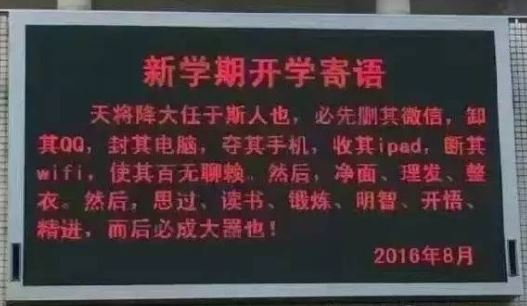4 2
2333 6666
CYLL 3 1234 2345 3456
U 4 9966 6666 8888 6666
GG 2 2333 7777
JJ 3 0012 6666 2333

U: 6666 6666
GG: 2333
JJ: 6666 2333
3 5

#!/usr/bin/env python
# -*-coding:utf-8 -*-
'''
@File    :   1072.py
@Time    :   2020/05/20 12:22:16
@Author  :   Schiller Xu
@Version :   1.0
@Desc    :   None
'''

n,m=map(int,input().split())
check_id=set()

#p存在问题的学生数，q被查的物品数
p=q=0
for i in input().split():
for i in range(n):
data=input().split()
s=''
for j in range(2,len(data)):
if data[j] in check_id:
q+=1
s=s+' '+data[j]
if s!='':
s=data+':'+s
p+=1
print(s)
else:
continue
print(p,q)


### 73、多选题常见计分法

3 4
3 4 2 a c
2 5 1 b
5 3 2 b c
1 5 4 a b d e
(2 a c) (3 b d e) (2 a c) (3 a b e)
(2 a c) (1 b) (2 a b) (4 a b d e)
(2 b d) (1 e) (1 c) (4 a b c d)

3.5
6.0
2.5
2 2-e
2 3-a
2 3-b

2 2
3 4 2 a c
2 5 1 b
(2 a c) (1 b)
(2 a c) (1 b)

5.0
5.0
Too simple

#!/usr/bin/env python
# -*-coding:utf-8 -*-
'''
@File    :   1073.py
@Time    :   2020/05/21 12:05:03
@Author  :   Schiller Xu
@Version :   1.0
@Desc    :   None
'''

n,m=map(int,input().split())
# test=[input().split() for i in range(m)]
#所有选项数据
#只有abc等选项
ans=[]
#错误选项
err_ch=dict()

for i in range(m):
data=input().split()
# for j in range(int(data)):
#     c=chr(97+j)
#     k=str(i+1)+'-'+c
#     err_ch[k]=0

for i in range(n):
data=input().strip('()')
data=data.split(') (')
#print(data)
score=0
for j in range(m):
#s=0
err=0
if rep==ans[j]:
else:
for r in rep:
if r not in ans[j]:
k=str(j+1)+'-'+r
try:
err_ch[k]+=1
except:
err_ch[k]=1
err+=1
#正确选项没被选上也算错误
for a in ans[j]:
if a not in rep:
k=str(j+1)+'-'+a
try:
err_ch[k]+=1
except:
err_ch[k]=1
if err==0:

#score+=s
print('{:.1f}'.format(score))

lens=len(err_ch)
if lens==0:
print('Too simple')
else:
max_err=max(err_ch.values())
result=[]
for k,v in err_ch.items():
if v==max_err:
r=[v,k,int(k[:-2]),k[-1]]
result.append(r)
result.sort(key=lambda x: (x,x))
for r in result:
print(r,r)


### 74、宇宙无敌加法器

30527
06203
415

7201

#!/usr/bin/env python
# -*-coding:utf-8 -*-
'''
@File    :   1074.py
@Time    :   2020/05/25 20:05:45
@Author  :   Schiller Xu
@Version :   1.0
@Desc    :   None
'''

string=input()
lens=len(string)

num_1=input()
num_2=input()
lens_1=len(num_1)
lens_2=len(num_2)
num_2='0'*(lens-lens_2)+num_2
num_1='0'*(lens-lens_1)+num_1

s=''
quotient=0
for i in range(lens-1,-1,-1):
#print(f's={s}')
sum=int(num_1[i])+int(num_2[i])+quotient
d=int(string[i])
try:
remain=sum%d
quotient=sum//d
except:
remain=sum%10
quotient=sum//10
s=str(remain)+s
if quotient!=0:
s=str(quotient)+s
print(int(s))


### 75、链表元素分类 (有超时)

00100 9 10
23333 10 27777
00000 0 99999
00100 18 12309
68237 -6 23333
33218 -4 00000
48652 -2 -1
99999 5 68237
27777 11 48652
12309 7 33218

33218 -4 68237
68237 -6 48652
48652 -2 12309
12309 7 00000
00000 0 99999
99999 5 23333
23333 10 00100
00100 18 27777
27777 11 -1

#!/usr/bin/env python
# -*-coding:utf-8 -*-
'''
@File    :   1075.py
@Time    :   2020/05/25 21:13:41
@Author  :   Schiller Xu
@Version :   1.0
@Desc    :   None
'''

data=input().split()
first=data
n=int(data)
k=int(data)

data=[]
next_=[]
# num_negative=0
# num_k=0
num_negative=[]
num_k=[]
num_others=[]

for i in range(n):
s=input().split()
data.append(s)
next_.append(s)

# result=[0 for i in range(n)]
result=[]

while first!='-1':
num=data[i]
if 0<=int(num)<=k:
# index_k+=1
elif int(num)<0:
# index_negative+=1
else:
# index_others+=1
first=next_[i]

result=num_negative+num_k+num_others

for i in range(len(result)-1):
print(result[i],result[i],result[i+1])
print(result[-1],result[-1],-1)


### 76、Wifi密码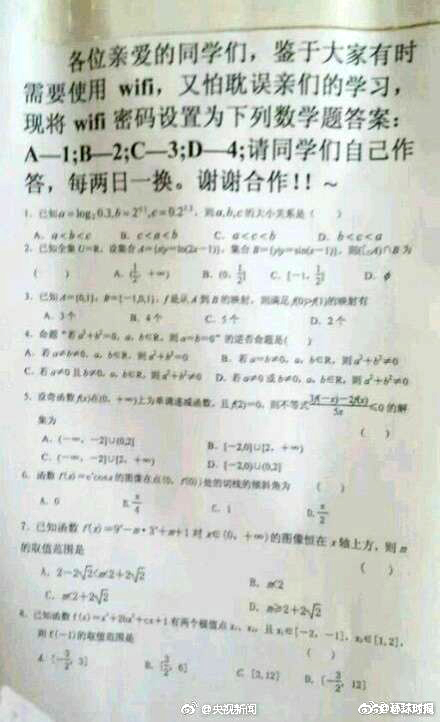8
A-T B-F C-F D-F
C-T B-F A-F D-F
A-F D-F C-F B-T
B-T A-F C-F D-F
B-F D-T A-F C-F
A-T C-F B-F D-F
D-T B-F C-F A-F
C-T A-F B-F D-F

13224143

#!/usr/bin/env python
# -*-coding:utf-8 -*-
'''
@File    :   1076.py
@Time    :   2020/05/27 15:51:50
@Author  :   Schiller Xu
@Version :   1.0
@Desc    :   None
'''

n=int(input())
s=''
check={'A':'1','B':'2','C':'3','D':'4'}

for i in range(n):
data=input().split()
for d in data:
if d[-1]=='T':
s=s+check[d]
break
print(s)


### 77、互评成绩计算

6 50
42 49 49 35 38 41
36 51 50 28 -1 30
40 36 41 33 47 49
30 250 -25 27 45 31
48 0 0 50 50 1234
43 41 36 29 42 29

42
33
41
31
37
39

#!/usr/bin/env python
# -*-coding:utf-8 -*-
'''
@File    :   1077.py
@Time    :   2020/05/27 16:03:19
@Author  :   Schiller Xu
@Version :   1.0
@Desc    :   None
'''

n,m=map(int,input().split())
for i in range(n):
data=[]
s=0
for j in input().split():
score=int(j)
if 0<=score<=m:
data.append(score)
g2=data
g1=0
data=data[1:]
data.sort()
for j in range(1,len(data)-1):
g1+=data[j]
s=(g1/(len(data)-2)+g2)/2
# print('{}')
s=str(s).split('.')
if len(s)==2 and int(s)>=5:
s=int(s)+1
else:
s=int(s)
print(s)


### 78、字符串压缩与解压

C
TTTTThhiiiis isssss a tesssst CAaaa as

5T2h4is i5s a3 te4st CA3a as

D
5T2h4is i5s a3 te4st CA3a as10Z

TTTTThhiiiis isssss a tesssst CAaaa asZZZZZZZZZZ

#!/usr/bin/env python
# -*-coding:utf-8 -*-
'''
@File    :   1078.py
@Time    :   2020/05/27 18:59:45
@Author  :   Schiller Xu
@Version :   1.0
@Desc    :   None
'''

c=input()
s=input()
if c=="C":
new_s=''
lens=len(s)
key_c=s
k=0
c_n=dict()
for j in range(lens):
if s[j]==key_c:
k+=1
else:
if k==1:
new_s+=key_c
else:
new_s+=str(k)+key_c
key_c=s[j]
k=1
if k==1:
new_s+=key_c
else:
new_s+=str(k)+key_c
else:
new_s=''
i=0
lens=len(s)
num=''
for j in range(lens):
if '0'<=s[j]<='9':
num+=s[j]
else:
if num!='':
num=int(num)
new_s+=s[j]*num
else:
new_s+=s[j]
num=''
print(new_s)


### 79、延迟的回文数

A + B = C

97152

97152 + 25179 = 122331
122331 + 133221 = 255552
255552 is a palindromic number.

196

196 + 691 = 887
887 + 788 = 1675
1675 + 5761 = 7436
7436 + 6347 = 13783
13783 + 38731 = 52514
52514 + 41525 = 94039
94039 + 93049 = 187088
187088 + 880781 = 1067869
1067869 + 9687601 = 10755470
10755470 + 07455701 = 18211171

#!/usr/bin/env python
# -*-coding:utf-8 -*-
'''
@File    :   1079.py
@Time    :   2020/05/28 15:17:01
@Author  :   Schiller Xu
@Version :   1.0
@Desc    :   None
'''

def check(num):
flag=True
num=str(num)
re_num=num[::-1]
if num!=re_num:
flag=False
return flag

a=input()
if check(a):
print(f'{a} is a palindromic number.')
else:
for i in range(10):
b=a[::-1]
c=int(a)+int(b)
print(f'{a} + {b} = {c}')
a=str(c)
if check(a):
print(f'{a} is a palindromic number.')
break
if i==9:


### 80、MOOC期终成绩

6 6 7
01234 880
a1903 199
ydjh2 200
wehu8 300
dx86w 220
missing 400
ydhfu77 99
wehu8 55
ydjh2 98
dx86w 88
a1903 86
01234 39
ydhfu77 88
a1903 66
01234 58
wehu8 84
ydjh2 82
missing 99
dx86w 81

missing 400 -1 99 99
ydjh2 200 98 82 88
dx86w 220 88 81 84
wehu8 300 55 84 84

p,m,n=map(int,input().split())

for i in range(p):
x,y=input().split()
if int(y)>=200:
for i in range(m):
x,y=input().split()
try:
except:
continue
for i in range(n):
x,y=input().split()
try:
if m>f:
else:
except:
continue

if g>=60:
print(g,g,g,g,g)


### 81、检查密码

5
123s
zheshi.wodepw
1234.5678
WanMei23333
pass*word.6

#!/usr/bin/env python
# -*-coding:utf-8 -*-
'''
@File    :   1081.py
@Time    :   2020/06/02 15:27:10
@Author  :   Schiller Xu
@Version :   1.0
@Desc    :   None
'''

n=int(input())

for i in range(n):
pw=input()
lens=len(pw)
if lens<6:
else:
num_point=0
num_letter=0
num_n=0
num_others=0
for c in pw:
if c=='.':
num_point+=1
elif 'a'<=c<='z' or 'A'<=c<='Z':
num_letter+=1
elif '0'<=c<='9':
num_n+=1
else:
num_others+=1
break
if num_others>0:
elif num_n==0 and num_letter>0:
elif num_n>0 and num_letter==0:
elif num_n>0 and num_letter>0:


### 82、射击比赛

ID x y


3
0001 5 7
1020 -1 3
0233 0 -1

0233 0001

#!/usr/bin/env python
# -*-coding:utf-8 -*-
'''
@File    :   1082.py
@Time    :   2020/06/02 15:58:07
@Author  :   Schiller Xu
@Version :   1.0
@Desc    :   None
'''

n=int(input())

for i in range(n):
name,x,y=input().split()
x=abs(int(x))
y=abs(int(y))



### 83、是否存在相等的差

8
3 5 8 6 2 1 4 7

5 2
3 3
2 2

#!/usr/bin/env python
# -*-coding:utf-8 -*-
'''
@File    :   1083.py
@Time    :   2020/06/03 09:49:32
@Author  :   Schiller Xu
@Version :   1.0
@Desc    :   None
'''

n=int(input())
data=[int(i) for i in input().split()]

result=dict()
for i in range(len(data)):
d=abs(data[i]-i-1)
try:
result[d]+=1
except:
result[d]=1

result=sorted(result.items(),key=lambda x: x,reverse=True)
for r in result:
if r>1:
print(r,r)


### 84、外观数列

d, d1, d111, d113, d11231, d112213111, …

1 8

1123123111

#!/usr/bin/env python
# -*-coding:utf-8 -*-
'''
@File    :   1084.py
@Time    :   2020/06/03 11:04:42
@Author  :   Schiller Xu
@Version :   1.0
@Desc    :   None
'''

d,n=input().split()
s=d
n=int(n)

for i in range(1,n):
key=s
k=0
new_s=''
for j in s:
if j==key:
k+=1
else:
new_s+=key+str(k)
key=j
k=1
new_s+=key+str(k)
s=new_s
print(s)


### 85、PAT单位排行 (有超时)

10
A57908 85 Au
B57908 54 LanX
A37487 60 au
T28374 67 CMU
T32486 24 hypu
A66734 92 cmu
B76378 71 AU
A47780 45 lanx
A72809 100 pku
A03274 45 hypu

5
1 cmu 192 2
1 au 192 3
3 pku 100 1
4 hypu 81 2
4 lanx 81 2

#!/usr/bin/env python
# -*-coding:utf-8 -*-
'''
@File    :   1085.py
@Time    :   2020/06/05 21:26:49
@Author  :   Schiller Xu
@Version :   1.0
@Desc    :   None
'''

n=int(input())

result=dict()

for i in range(n):
test_id,score,school=input().split()
school=school.lower()
k=test_id
if k=='B':
score=int(score)/1.5
elif k=='A':
score=int(score)
else:
score=int(score)*1.5
try:
result[school]+=score
result[school]+=1
except:
result[school]=[score,1]

for v in result.values():
v=int(v)
result=sorted(result.items(),key=lambda x: (-x,x,x))
print(len(result))

rank=1
print(rank,result,result,result)
for i in range(1,len(result)):
if result[i]!=result[i-1]:
rank=i+1
print(rank,result[i],result[i],result[i])


### 86、就不告诉你5 7

53

#!/usr/bin/env python
# -*-coding:utf-8 -*-
'''
@File    :   1086.py
@Time    :   2020/06/09 15:43:23
@Author  :   Schiller Xu
@Version :   1.0
@Desc    :   None
'''

a,b=map(int,input().split())
result=str(a*b)
# result=result[::-1]
# print(result)
s=''
for i in range(len(result)-1,-1,-1):
s+=result[i]
s=int(s)
print(s)


### 87、有多少不同的值

2017

1480

#!/usr/bin/env python
# -*-coding:utf-8 -*-
'''
@File    :   1087.py
@Time    :   2020/06/09 19:18:53
@Author  :   Schiller Xu
@Version :   1.0
@Desc    :   None
'''

n=int(input())
#s=n-int(n/6)-int(n/10)-int(n/15)+2*int(n/30)
s=n//2+n//3+n//5-n//6-n//10-n//15+n//30+1
print(s)


### 88、三人行

48 3 7

48 Ping Cong Gai

48 11 6

No Solution

#!/usr/bin/env python
# -*-coding:utf-8 -*-
'''
@File    :   1088.py
@Time    :   2020/07/01 16:07:52
@Author  :   Schiller Xu
@Version :   1.0
@Desc    :   None
'''

#甲是两位数，一个一个试过去就行
def compare(m,n):
if m==n:
return 'Ping'
elif m>n:
return 'Gai'
else:
return 'Cong'

m,x,y=map(int,input().split())
for i in range(99,9,-1):
#a、b、c分别表示甲乙丙
a=i
b=int(str(a)[::-1])
c=abs(a-b)/x
if b==c*y:
print(a,compare(m,a),compare(m,b),compare(m,c))
exit()
print('No Solution')

03-04
01-2864
07-28647
03-22397
04-07165
03-3171
10-24281
03-22208
02-27246
08-192968
03-20566
10-03895
07-04900
08-20320
05-08899
05-191246
03-16126
02-17621
03-131271
02-17452

### “相关推荐”对你有帮助么？

•非常没帮助
•没帮助
•一般
•有帮助
•非常有帮助被折叠的  条评论 为什么被折叠?到【灌水乐园】发言¥2 ¥4 ¥6 ¥10 ¥20余额支付 (余额：-- )扫码支付获取中扫码支付点击重新获取扫码支付1.余额是钱包充值的虚拟货币，按照1:1的比例进行支付金额的抵扣。
2.余额无法直接购买下载，可以购买VIP、C币套餐、付费专栏及课程。余额充值